# Paulo Freire's Polytopes

Hi, welcome to my office at the Max Planck Institute for Radio Astronomy, in Bonn!

First, let's have a look around!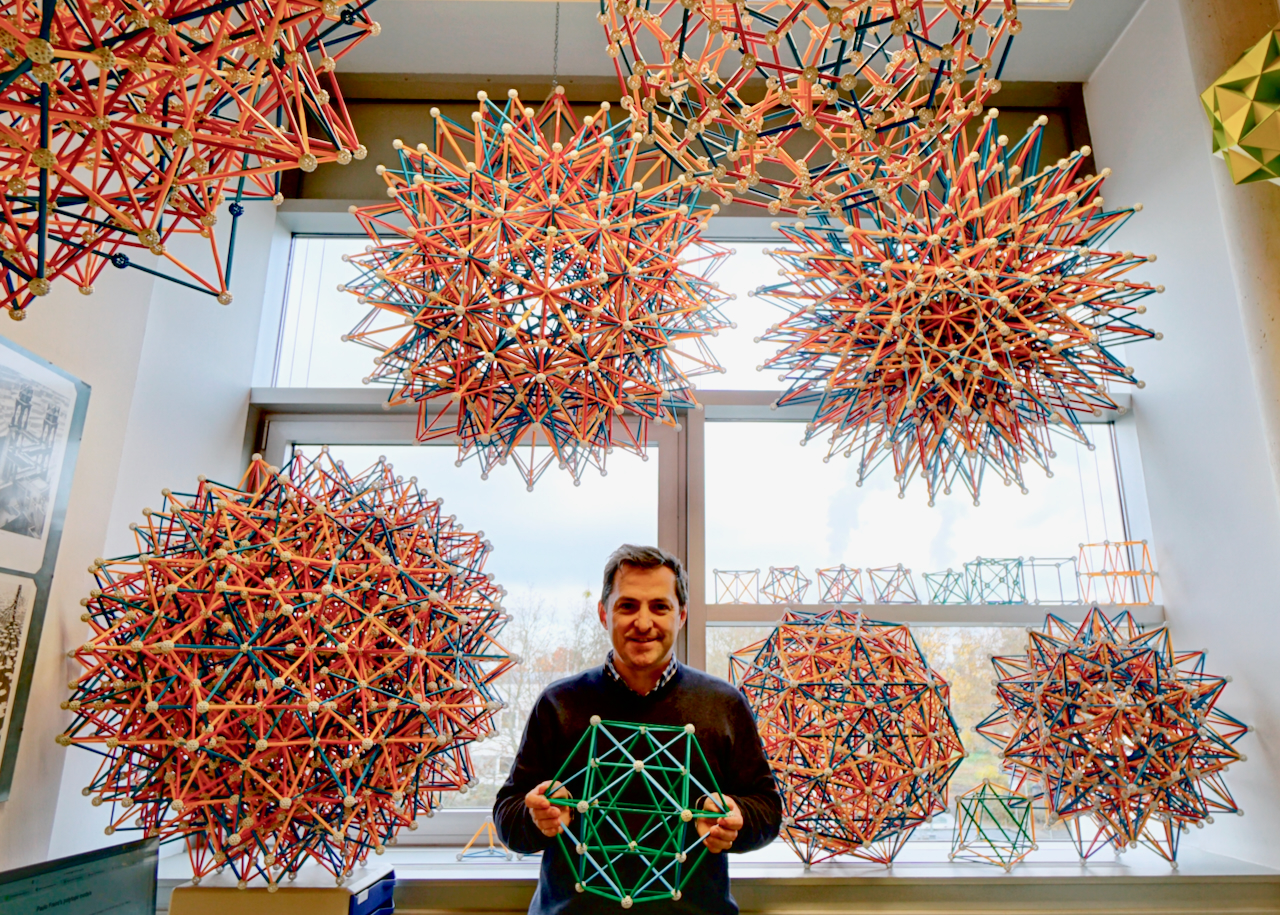Fig. 1a: This is me in my polytope garden, holding a model of an orthographic projection of the 600-cell to 3 dimensional space. Photo by Aris Noutsos.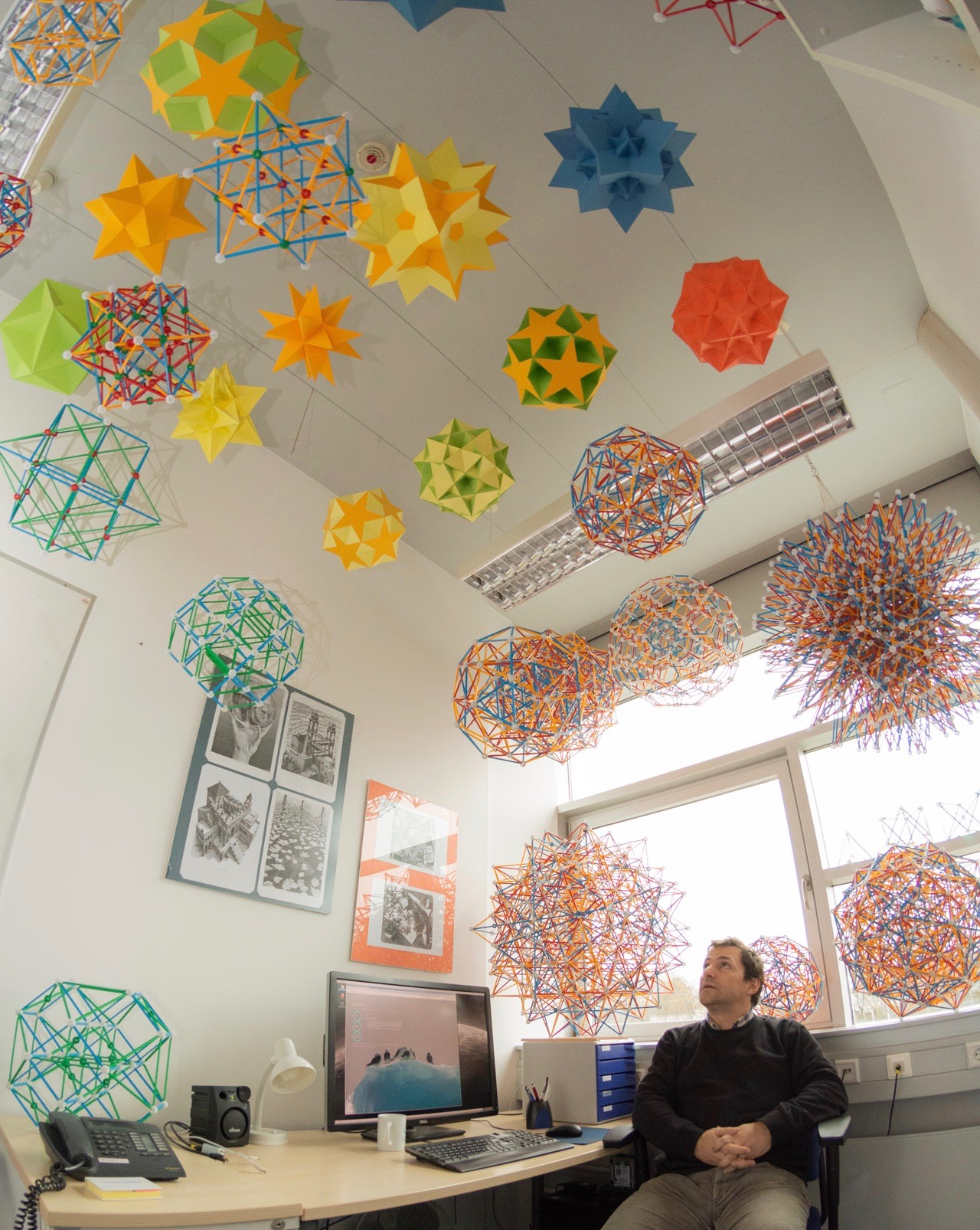Fig. 1b: A wide view of the office. Photo by Aris Noutsos.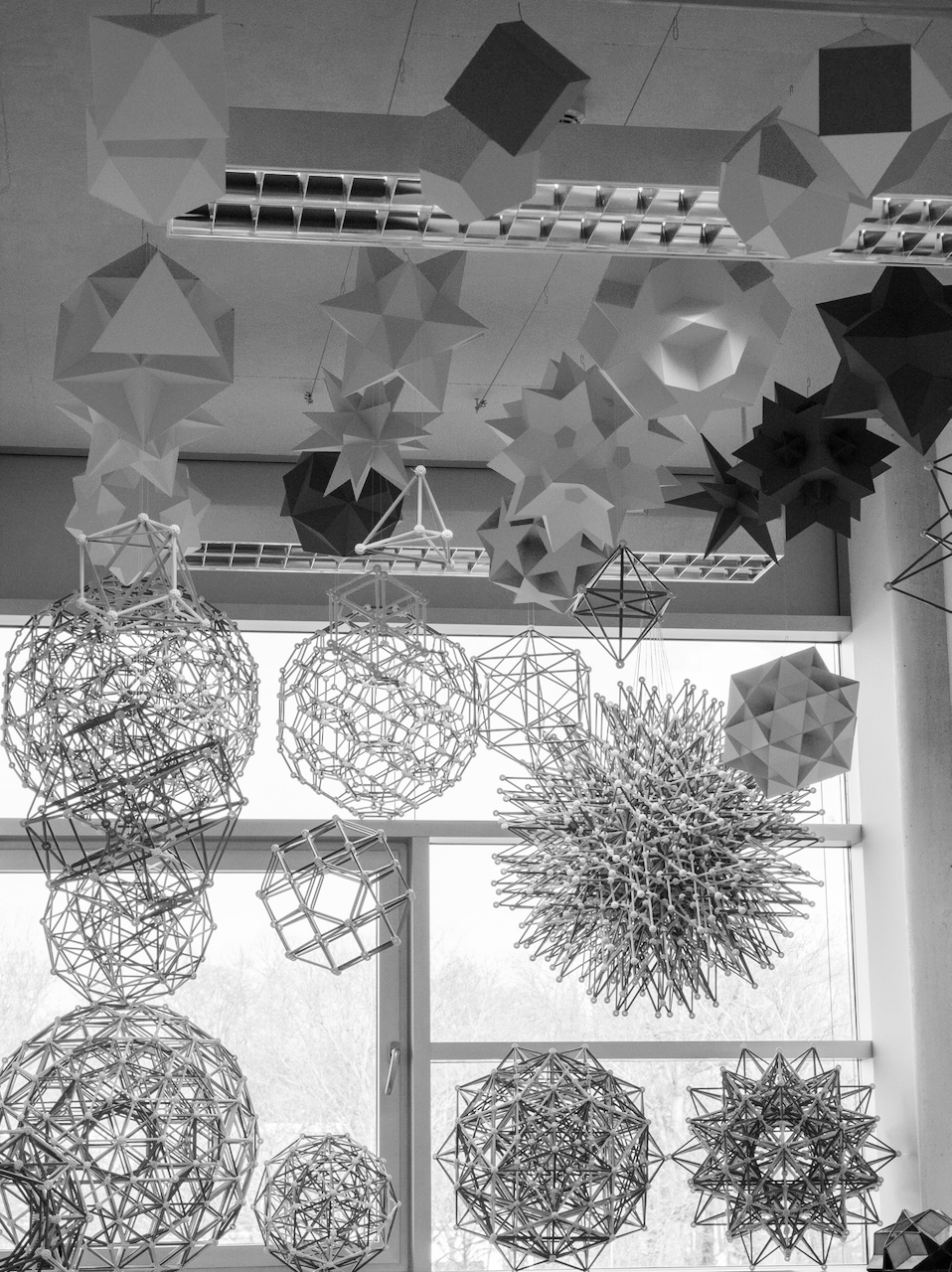Fig. 1c: A narrower view towards some of the most complex models of 4-dimensional polytopes, taken in 2018. Photo by Aris Noutsos.

### Why polytopes?

A few decades ago, I started noticing polyhedra, partly because of Carl Sagan's Cosmos, but also because of the art of M. C. Escher. What attracted me to them was first and foremost their pure, symmetric beauty. This is a cold and austere beauty possessed by all things necessary - things that were not invented, that have nothing arbitrary or left to chance, that are the way they are and cannot logically be anyway else, things that are complete and yet have nothing unnecessary - to use another word, of things perfect. Mathematics is where most such things exist; it is the realm of what is, to me, the highest form of beauty.

Polyhedra, and more generally polytopes are, among mathematical concepts, one of the easiest to understand and visualize. This makes them a gateway to that higher world of order and beauty which lies beyond our own.

This special feature of polyhedra is what motivated me to build these models. In the late 1990's - early 2000's I built a significant number of models of Uniform polyhedra; these are in my parents' country house, in Portugal. The recent models, built since 2009 and currently in my office, are the topic of this page; these include (since 2014) 3-D shadows of the 4-dimensional analogues of polyhedra, the polychora; these were built using the Zometool system.

News on the Zometool models:
2019 January 26: built model of Gosset's 8-dimensional figure. This was later disassembled.
2018 December 19: completed the compound of 15 16-cells.
2018 August 4: Completed the compound of 5 24-cells.
2017 November 11: Completed the Great grand stellated 120-cell. This completes the list of projections of all 16 regular polychora.
2017 November 2: Some of my models are being displayed at the Gutenberg store in Bad Godesberg. See poster here by Ⓒ Zometool Europa / Lyn Taylor, with photos by Aris Noutsos and Jason Wu.
2016 November 12: Completed larger scale version of the rectified 120-cell. Later disassembled.
2016 February 13: Completed rectified 600-cell. Later disassembled.
2015: Moved to new office.
2014 August 21: Completed model of the Grand 600-cell.
2014 August 6: Completed model of the stellated 120-cell.

 Geometric models in my office: - 3-D paper models: Regular and Quasi-regular Polyhedra - 3-D Zometool models - The fourth dimension: Regular and Uniform Polychora Convex Regular and Uniform Polychora    Non-convex Regular Polychora - Regular polychoron compounds - References Related pages in this site: - My old collection of Uniform polyhedra - The many stellations of the Rhombic triacontahedron - Beyond the fourth dimension

## Regular and Quasi-regular Polyhedra

``Without Geometry life is pointless.''
In ``King of Infinite Space: Donald Coxeter, the Man Who Saved Geometry'', by Siobhan Roberts.

From 2009 to 2013, I built a small collection of geometric paper models for my office, which includes a few of the uniform polyhedra and their duals:
First, a short explanation of the meaning of duality. For each face of a polyhedron, there is a perpendicular line from the center of the dual polyhedron to the dual's vertex. For each edge of a polyhedron, the dual polyhedron has a perpendicular edge. The duality operation transforms the face of a polyhedron into the vertex figure of its dual, and vice-versa. This is very important for understanding what follows.

The sixteen regular and quasi-regular polyhedra have profound similarities since they are uniform polyhedra sharing the same Wythoff construction, and thus have similar Wythoff symbols: Their vertices are reflections of one of the 3 vertices of the Schwarz triangle, the fundamental domain of their corresponding 3-D point symmetry group. All of these polyhedra are isotoxal, i.e., they are edge-transitive. By the rules of duality, this applies to their duals as well.

Different Wythoff constructions would result in other types of uniform polyhedra, like the remaining Archimedean polyhedra (which include the Truncated icosahedron that everyone asks about when they come to my office - no, I don't have that model in my office). Apart from the quasi-regulars, none of the others is isotoxal.

For the following pictures of polyhedra, the color code is as follows: the non-regular faces are blue, they belong to the duals of the quasi-regular polyhedra; for the regular faces, the equilateral triangles (henceforth Triangles) are yellow, squares (henceforth Squares) are red, regular pentagons (Pentagons) are green and regular pentagrams (Pentagrams) are gold. Since the latter are regular polygons, the polyhedra built from them shown below are either regular or quasi-regular. Thus, when we discuss e.g., the regular tetrahedron, we use simply the capitalized word Tetrahedron, and likewise for Octahedron, Icosahedron and Dodecahedron.Fig. 2: The convex polyhedron models. Bottom row, from right to left: 1 - The Tetrahedron (the only model that has tetrahedral symmetry), 2 - its rectification, the Octahedron, 3 - the dual of the Octahedron, the Cube, 4 - the rectification of the Cube and Octahedron - the Cuboctahedron and 5 - the dual of the Cuboctahedron, the Rhombic dodecahedron (these last four have octahedral symmetry). Top row, right to left: 1 - the Icosahedron and 2 - its dual, the Dodecahedron, 3 - the rectification of the Icosahedron and the Dodecahedron - the Icosidodecahedron and 4 - the dual of the Icosidodecahedron, the Rhombic triacontahedron (these four have icosahedral symmetry). The five polyhedra on the right (Tetrahedron, Octahedron, Cube, Icosahedron and Dodecahedron) are the five platonic polyhedra, the only regular convex polyhedra. The Cuboctahedron and Icosidodecahedron (those with two colors) are the only convex quasi-regular polyhedra, and the two rhombic polyhedra in blue are their duals.

Given the way it was built, the Cuboctahedron has the set of faces of the Cube and of the Octahedron - six Squares and eight Triangles. This means that its dual, the Rhombic dodecahedron, has the 6 vertices of the Octahedron (where four edges meet) and the 8 vertices of the Cube (where three edges meet). The same applies to the Icosidodecahedron, which has the 20 triangular faces of the Icosahedron and the 12 pentagonal faces of the Dodecahedron. Its dual, the Rhombic triacontahedron, has the 12 vertices of the Icosahedron (where 5 edges meet) and the 20 vertices of the Dodecahedron, where 3 edges meet.
This picture includes the full set of convex isotoxal polyhedra.

Frame of picture taken by Jason Wu.

A few notes on the non-convex polyhedra that follow (Figs. 3 - 6):
1. They all have icosahedral symmetry,
2. They are either regular or quasi-regular or duals of the latter, which makes all of them isotoxal,
3. The vertices of all regular and quasi-regular polyhedra, like all uniform polyhedra, belong to a sphere that circumscribes the whole polyhedron and is concentric with it. The inner vertices are false: several edges intersect there, but none ends there. In what follows, we generally refer only to the true vertices.
4. These vertices are arranged as either the Dodecahedron, Icosahedron or Icosidodecahedron, i.e., they are facetings of these convex polyhedra which are therefore their convex hulls. By a general rule, their dual polyhedra are stellations of the duals of their convex hulls.

The reason for this is simple: take line from a vertex to the center of polyhedron A, which is convex. The face of the dual polyhedron (B) is perpendicular to this line. Since we must keep the same vertex arrangement for any faceting of A, then the faces of its dual must still lie in the same perpendicular planes of the faces of B - i.e, the dual of the faceting of A must be a stellation of B. This is a very important rule, which we must keep in mind in order to understand what follows.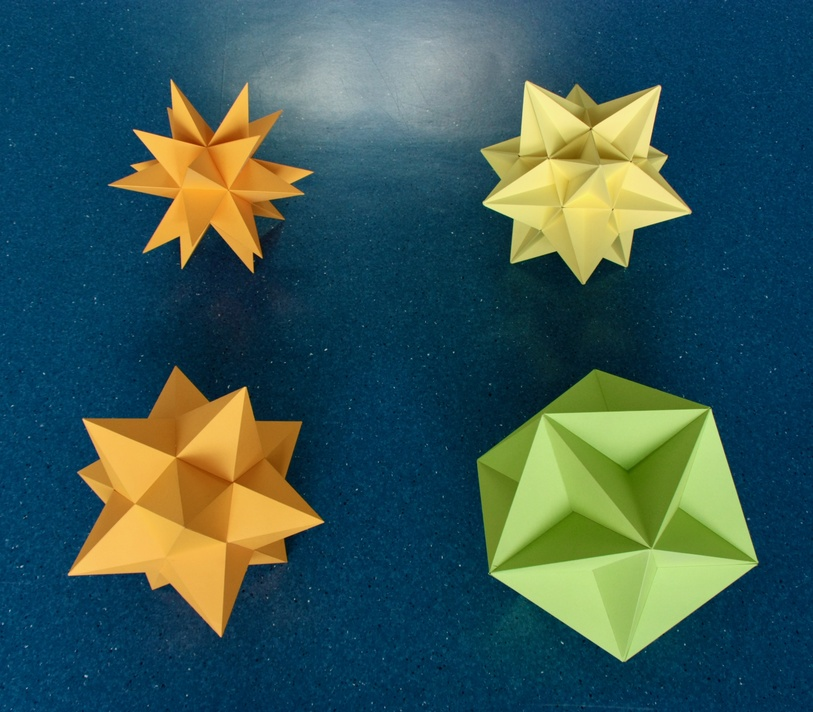Fig. 3: The four Kepler-Poinsot polyhedra: the Small stellated dodecahedron (top left) and its dual, the Great dodecahedron (bottom left), the Great stellated dodecahedron (top right) and its dual, the Great icosahedron (bottom right).

Frame of picture taken by Jason Wu.

The relations between the Dodecahedron, Icosahedron and the Kepler-Poinsot polyhedra are displayed in Figs 4a and 4b below.

Following Coxeter (1973), we first arrange these six polyhedra as the vertices of an imaginary regular Hexagon. The vertical scale indicates the density. Duality is given by the two-sided yellow arrows.
Coxeter then highlights the fact that each line going through the center of this Hexagon (here in red) changes a pentagon into a pentagram, or vice-versa, either in the faces or in the vertex figures of the polyhedra at the ends of the line. This results in isomorphic polyhedra. This means that the polyhedra at the ends of the red lines are topologically identical: they have the same number of faces, edges and vertices; the faces have the same number of sides, and the vertices have the same number of faces and edges converging into them, etc.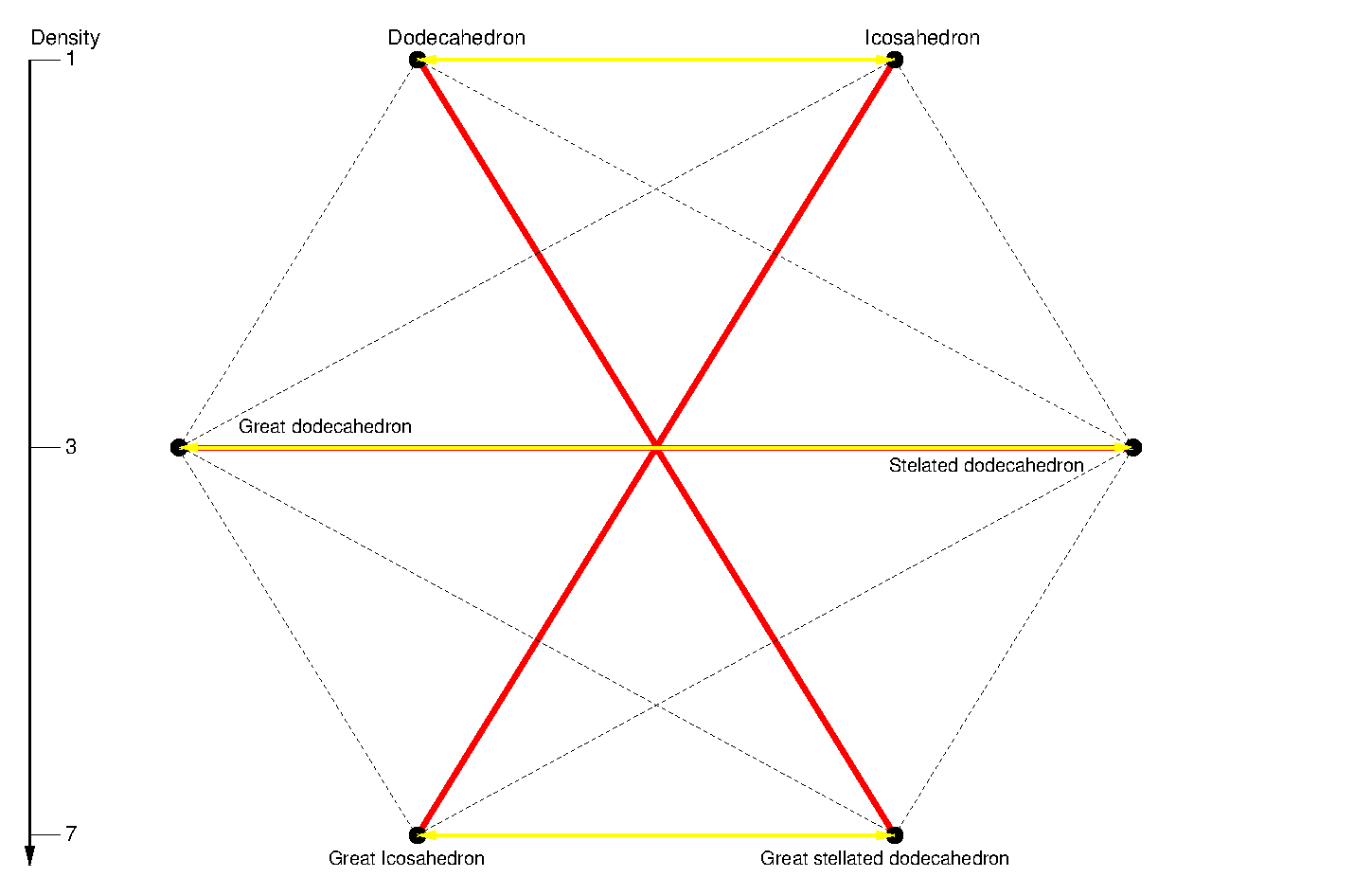Fig. 4a: Geometric relationships between the Dodecahedron, Icosahedron and the Kepler-Poinsot polyhedra. The yellow arrows denote duality among two polyhedra. The red lines show isomorphic transformations between two polyhedra.

This hexagonal arrangement is also useful for highlighting other geometric relationships among polyhedra, which can be represented by sets of parallel lines. There are two ``stellation'' operations, that extend the faces of a particular polyhedron. The first, edge stellation (represented as cutting through the Hexagonal arrangement, not through the edges or through the center), extends the edges of the faces (obviously in the same direction, but both ways) until they meet again, this is illustrated in Fig. 4b by the blue arrows. This can only start from polyhedra with pentagonal faces. The second operation, Greatening, replaces a face with a larger one of the same kind in the same plane; this is represented by the green arrows.

Both operations conserve the number of faces and their planes; therefore all polyhedra derived from the Dodecahedron (Great dodecahedron, Small stellated dodecahedron and Great dodecahedron) - the full set of stellations of the Dodecahedron - have 12 faces, as their names indicate. They are represented in Fig. 11b, which also shows how they can be derived geometrically by the process of extending the planes of the faces. The Great icosahedron, being a greatening of the Icosahedron, has 20 triangular faces. It is the only of the 59 stellations the Icosahedron that is regular.

In Fig. 4b we also present the faceting, or ``conservation'' operations, which as we describe below are the duals of the stellation operations. The solid red lines indicate faceting with conservation of edge arrangement: Indeed, the Great dodecahedron has the same edge arrangement as the Icosahedron, and the Great icosahedron has the same edge arrangement of the Small stellated dodecahedron, as can be seen in Fig. 3. This operation facets the pentagonal vertex figure into a pentagram. The dashed lines indicate faceting with conservation of the type of vertex figure, but reduced in angular size. Both the solid red lines and the dashed lines imply conservation of the vertex arrangement, which all faceting operations must obey by definition.

It follows from these conservation laws that the four polyhedra in the red circuit must share the same vertex arrangement - in this case of the Icosahedron. This means that they are facetings of the Icosahedron (see point number 3 above when introducing the non-convex polyhedra); they are represented in Fig. 11c. The two polyhedra in the pink dotted line have the vertex arrangement and vertex figure of the Dodecahedron.
Why these conservation relations exist is explained below.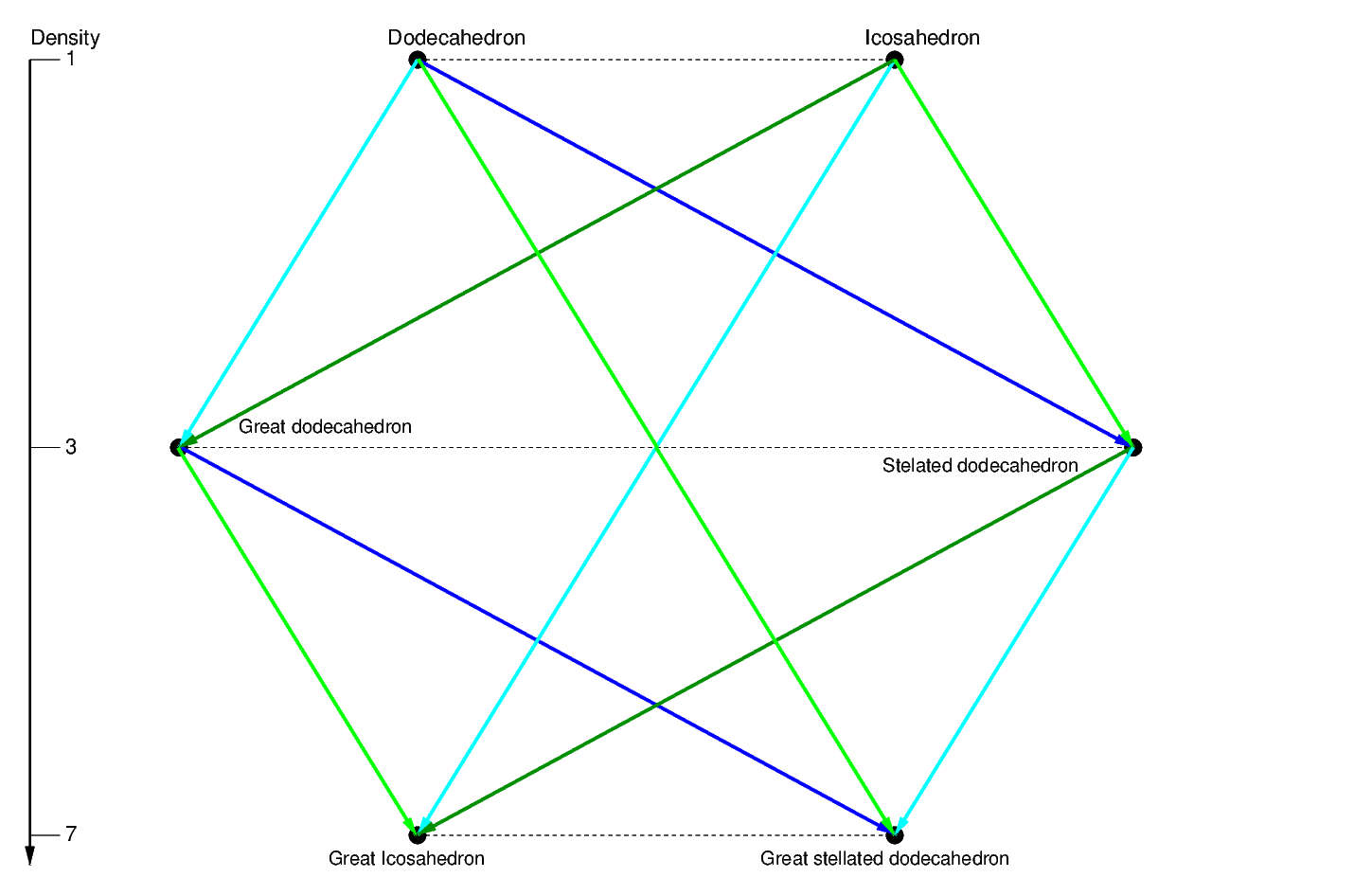Fig. 4b: Relations between the Dodecahedron, Icosahedron and the Kepler-Poinsot polyhedra.

(Technical bit: please skip if you're devoid of curiosity).
Why do these conservation relations exist? The answer is because they can be described as duals to the stellation operations. In particular:
1) Conservation of edges (red) = find dual + edge stellate + find dual
2) Conservation of vertex figure (dotted) = find dual + greaten + find dual

As an example of 1), let's move from the Icosahedron to the Great dodecahedron along the solid red line. This is the same as finding the dual of the Icosahedron (Dodecahedron), edge stellating (Small stellated dodecahedron) and finding the dual of the latter (Great dodecahedron). The first operation transformed the edges of the Icosahedron into the perpendicular edges, those of the Dodecahedron. The second operation merely extended those edges, but kept their directions. The last operation find edges perpendicular to those of the Small stellated dodecahedron - those of the original Icosahedron!
So what exactly does this edge-conservation operation do? The answer is to facet the vertex figure. The vertex figure of the Icosahedron is a Pentagon, the vertex figure of the Great dodecahedron is the inscribed Pentagram. This is fitting, as its dual operation stellates the faces.

As an example of 2), let's move from the Dodecahedron to the Great stellated dodecahedron along the dotted pink line. This is the same as finding the dual of the Dodecahedron (Icosahedron), greatening (Great icosahedron) and finding the dual of the latter (Great stellated dodecahedron). The first duality converts the triangular vertex figure of the Dodecahedron into the triangular face of the Icosahedron. Then greatening to a Great icosahedron keeps the same type of face, but on a larger scale (as greatening always does). In the final dual transformation, the triangular faces of the Great icosahedron are converted back to triangular vertex figures, as in the original Dodecahedron, but one smaller angular scale. Thus, this operation merely shrinks the vertex figure.

All faceting operations preserve the vertex arrangements. This is because their dual (the stellation operations) preserve the planes of the faces.
(end of technical bit)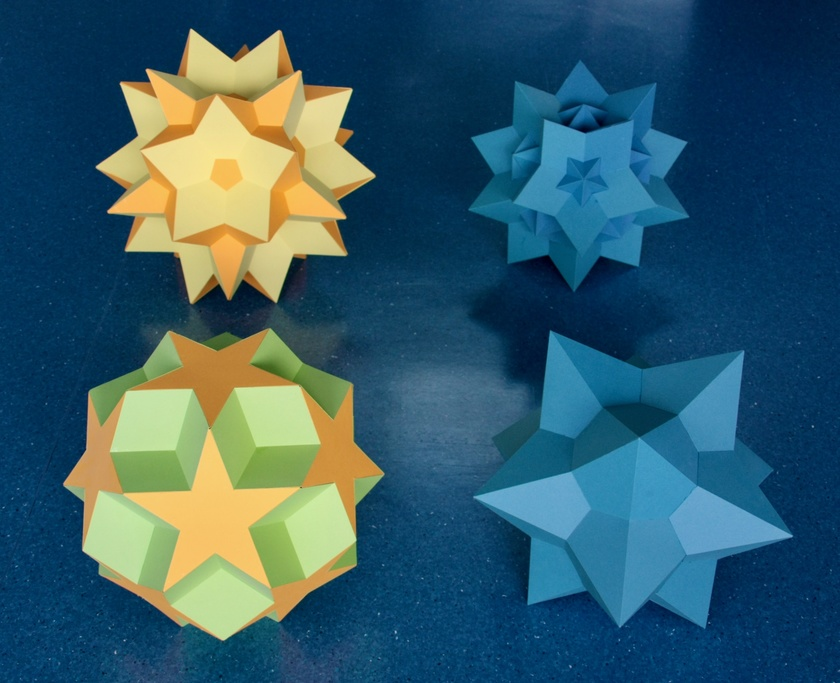Fig. 5: Two quasi-regular star polyhedra and their duals. Top right: the rectification of the Great dodecahedron and the Small stellated dodecahedron, a polyhedron that contains the 12 pentagonal faces of the former and the 12 pentagrammic faces of the latter - the Dodecadodecahedron. Bottom right: its dual, the Medial rhombic triacontahedron.
Top left: the rectification of the Great icosahedron and the Great stellated dodecahedron, a polyhedron that contains the 20 triangular faces of the former and the 12 pentagrammic faces of the latter - the Great icosidodecahedron. Bottom left: its dual, the Great rhombic triacontahedron.
The operation of rectification corresponds to finding the middle of the edges of a polyhedron and transforming it in a new vertex. Doing this for any two dual polyhedra results in the same polyhedron, since their edges are perpendicular and intersect at their midpoints.

Rectifying the Kepler-Poinsot polyhedra, we always obtain the vertex arrangement of the Icosidodecahedron. We can see this easily from Fig. 4b: the Small stellated dodecahedron can be obtained from the Dodecahedron by a symmetric (both ways) edge stellation. Thus the arrangement of the mid-points of its edges remained unchanged under edge stellation - it is still the arrangement of the vertices of the Icosidodecahedron. The same necessarily applies to its dual, the Great dodecahedron.
The Great stellated dodecahedron can similarly be obtained from the Great dodecahedron by edge stellation, therefore the mid-points of its edges still have the same arrangement as the Icosidodecahedron. The same will necessarily apply to its dual, the Great icosahedron.

Since the rectifications of the Kepler-Poinsot polyhedra (the Dodecadodecahedron and the Great icosidodecahedron in the top row) are facetings of the Icosidodecahedron, their duals must be stellations of the dual of the Icosidodecahedron, the Rhombic triacontahedron.

One final note: as we have seen, the Great Icosahedron is topologically identical to the Icosahedron. For this reason, the Great icosidodecahedron, the rectification of the Great icosahedron, is topologically identical to the Icosidodecahedron, the rectification of the Icosahedron.

Frame of picture taken by Jason Wu.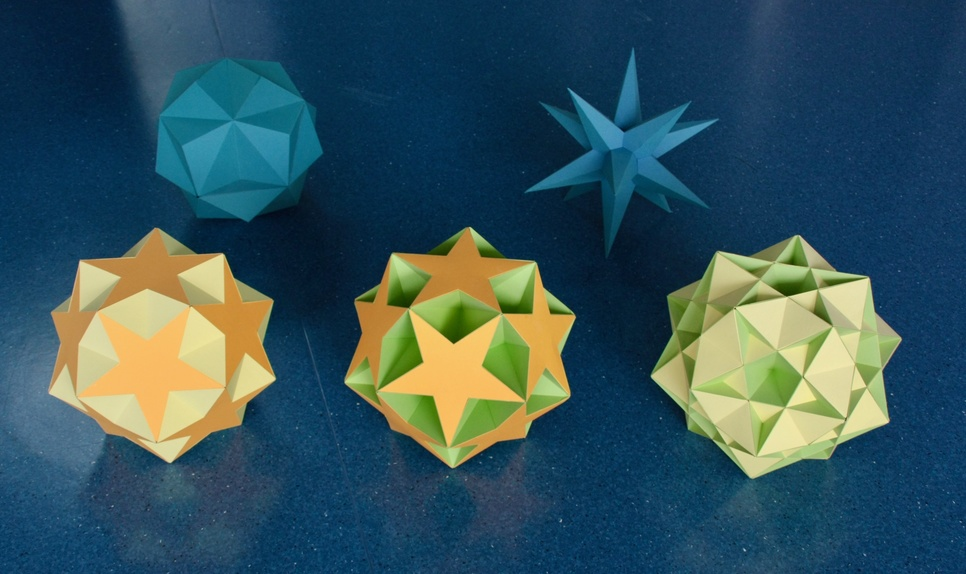Fig. 6: We now show a few of the facetings of the Dodecahedron, in particular the ditrigonal quasi-regular star polyhedra: The Small ditrigonal icosidodecahedron (left) and its dual, the Small triambic icosahedron (center left, in blue), the Ditrigonal dodecadodecahedron (center right) and its dual, the Medial triambic icosahedron (right, in blue), the Great ditrigonal icosidodecahedron (far right, in the foreground) and its dual, the Great triambic icosahedron (right, in blue) - this is represented by the same model as the Medial triambic icosahedron as they only differ in their internal structure. Since the ditrigonal star polyhedra are facetings of the Dodecahedron, their duals are, of course, stellations of the Icosahedron.

The three ditrigonal quasi-regular polyhedra all share the edge arrangement of the compound of five cubes. The edges of their duals (in blue) (and the dual of the compound of five cubes, the compound of five octahedra) are perpendicular to the former, which means that they are all have the same directions - indeed, they can all be derived from the first stellation of the Icosahedron (the Small triambic icosahedron) by edge stellation.

Picture taken by Aris Noutsos.

Not all polyhedron models in my office are made of paper, as shown by the next few figures.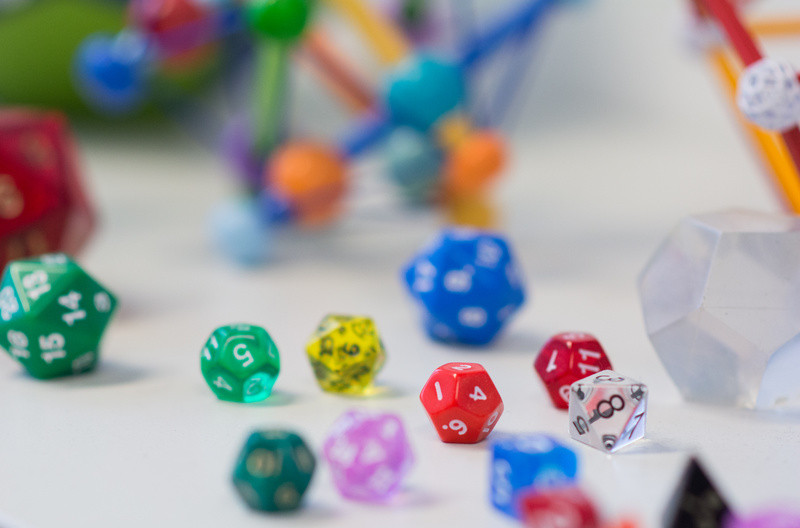Fig. 7a: Here are the platonic polyhedra, this time as dice. Picture by Aris Noutsos.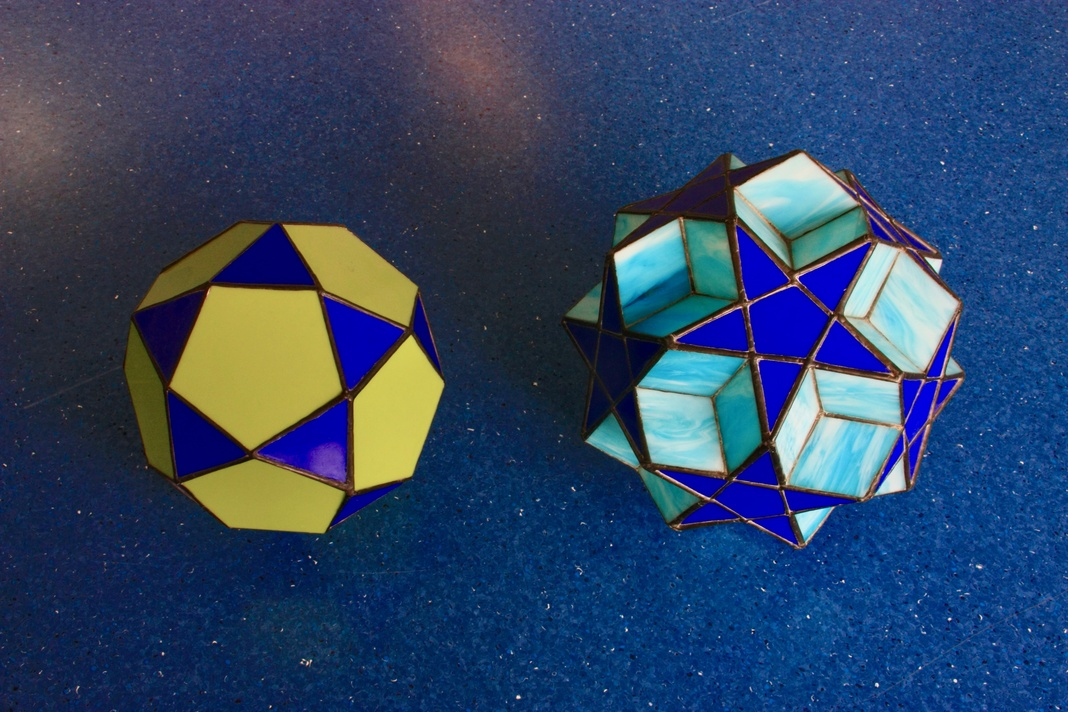Fig. 7b: Here are stained glass models of the Icosidodecahedron (right) and of the Dodecadodecahedron (left). Made by Naomi Janches.

Before ending this section, it is important to remark that the regular and quasi-regular polyhedra shown above represent only a small fraction of the Uniform polyhedra. For more models of the latter, check my page on Uniform polyhedra and also Fig. 8 below.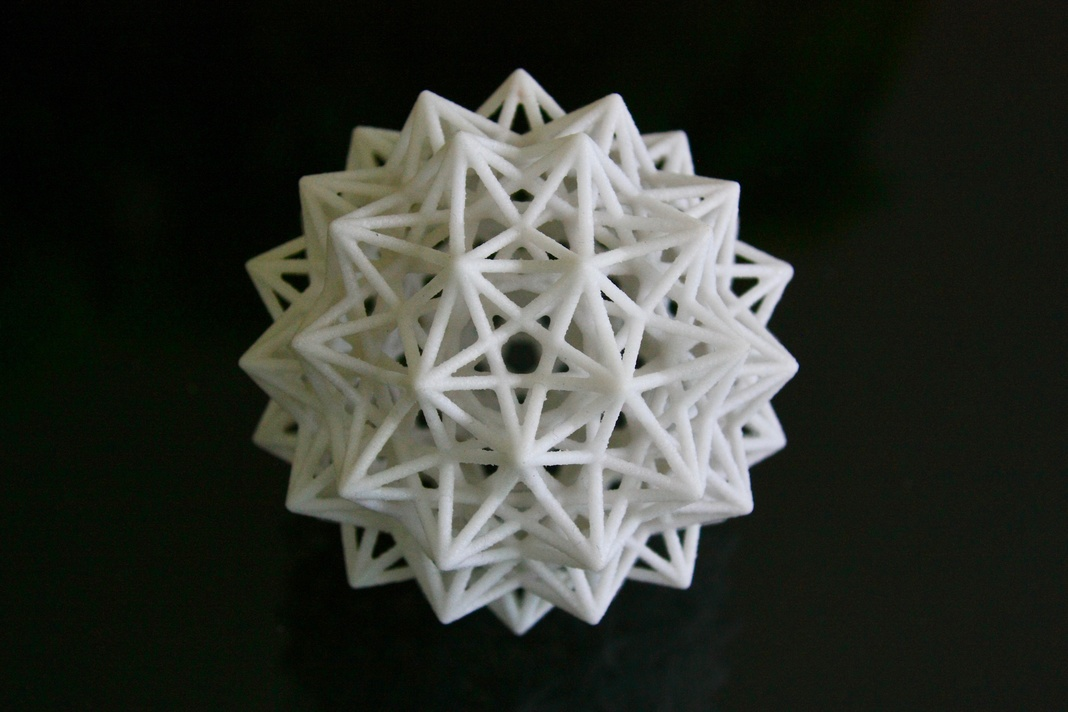Fig. 8: Here is a 3-D printed edge model of a uniform polyhedron compound, the compound of 20 Octahedra.

This set of edges is shared by two remarkable uniform polyhedra. The first is the Great dirhombicosidodecahedron, a uniform polyhedron with a highly unusual Wythoff construction. For this reason it is also known as ``Miller's Monster". This was the last entry in the 1954 list of uniform polyhedra compiled by J. C. P. Miller, H. S. M. Coxeter and M. S. Longuet-Higgins.

The other remarkable polyhedron that shares this set of edges is the Great dis-nub dirhombidodecahedron. The latter object was discovered in 1975 when John Skilling proved that the 1954 list of uniform polyhedra is complete. In that paper he generalized the concept of Uniform polyhedron: instead of two faces per edge, there can be any even number (or, equivalently, edges can be multiple). This generalization adds only this new figure, for that reason this it is also known as ``Skilling's figure".

Finally, the edges also belong to another special uniform polyhedron compound, the Compound of twenty tetrahemihexahedra.

Model printed by Shapeways, design by Mathematical Creations.

## 3-D Zometool models

``Thus, the chief reason for studying regular polyhedra is still the same as in the time of the Pythagoreans, namely, that their symmetrical shapes appeal to one's artistic sense.''
H. S. M. Coxeter, in the preface to ``Regular Polytopes''.

In 2014 I started building Zometool models. Such models display only the edge arrangements of a geometrical object. Therefore, they cannot display face arrangements. A consequence of this is that the Icosahedron and the Great dodecahedron, which share the same edge arrangements, are represented by the same Zometool model. The same would happen for the Small stellated dodecahedron and the Great icosahedron. The three ditrigonal quasi-regular polyhedra in Fig. 6 and the compound of five cubes are all represented by the same Zometool model (see below).

The main advantage of Zometool models, however, is that they allow the display of internal structure; for the models that follow that is absolutely necessary. As an example, in the Zometool model below I display a model that shows many geometric relations between the Platonic polyhedra. This particular kit was extremely useful for me to familiarize myself with the extremely interesting properties of Zometool, something that I suggest the reader does before attempting to build any of the models that follow.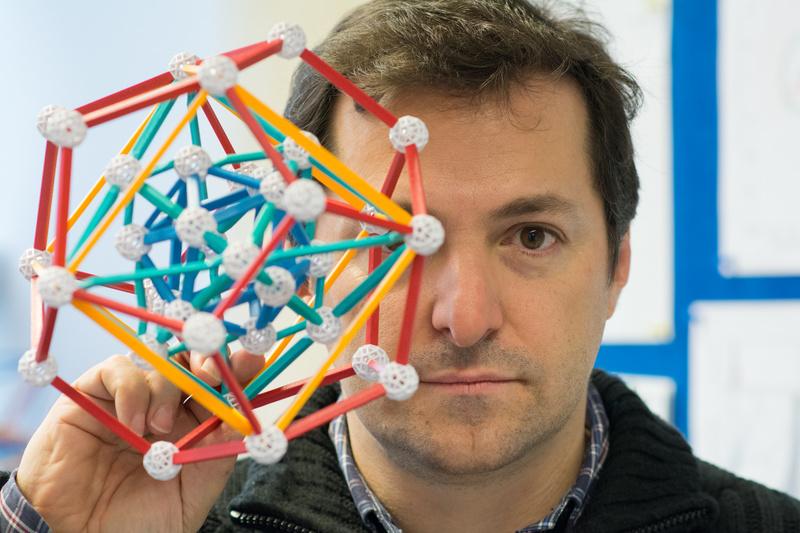Fig. 9: Myself holding a beautiful geometrical model that illustrates many interesting relations between the Platonic polyhedra and their symmetries. This is sold by Zometool as Kepler's Kosmos, and was designed originally by John H. Conway. Photo by Aris Noutsos.

The models below represent stellations of the five convex isohedral polyhedra in Fig. 2 that can be stellated. This set consists of 3 Platonic polyhedra (the Octahedron, Dodecahedron and Icosahedron) plus the Rhombic dodecahedron and Rhombic triacontahedron. Being isohedral, they have only one stellation diagram; this makes the model much easier to plan and results in more appealing models. We also present facetings of the Cube, Icosahedron and Dodecahedron; however the latter models are not permanently in my office for lack of space.
Among these stellations and facetings we find many of the polyhedra shown above plus some interesting regular polyhedron compounds.

Note: Several of these models use non-standard, extra-long Zometool parts. These can be built with regular parts with a ball and two struts, but the results are not so satisfying. If you'd like to acquire extra-long parts, I suggest purchasing old Zometool sets on eBay.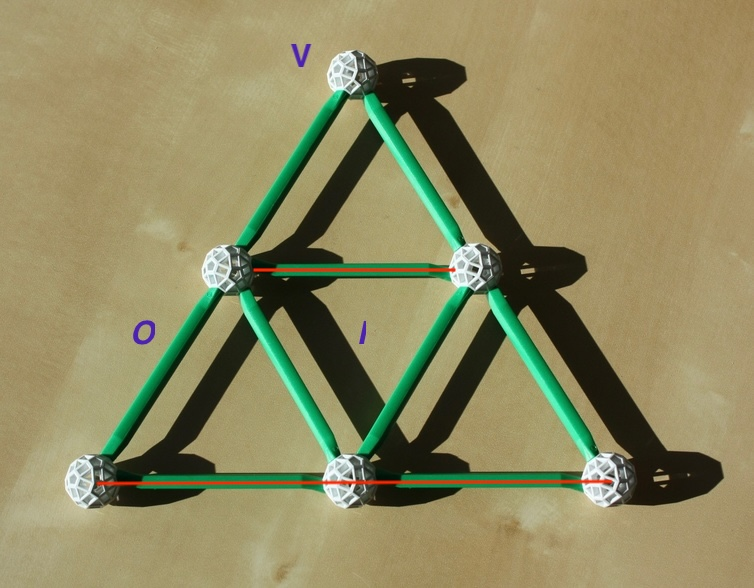Fig. 10a: This is the stellation pattern of the Octahedron. The inner triangle is the face of the Octahedron. The outer triangle is the face of its only stellation, known as the Stella Octangula.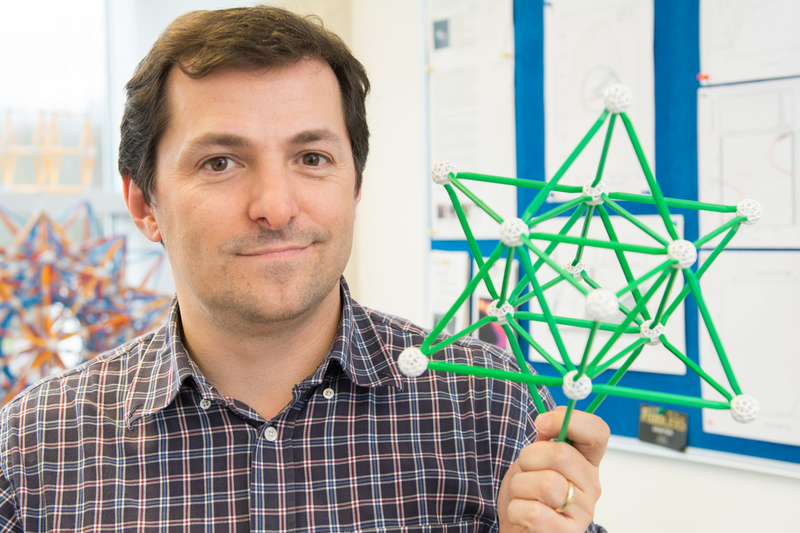Fig. 10b: Myself holding a model of the Stella Octangula. Here we can see that this stellation can be seen as an intersection of two Tetrahedra. Photo by Aris Noutsos.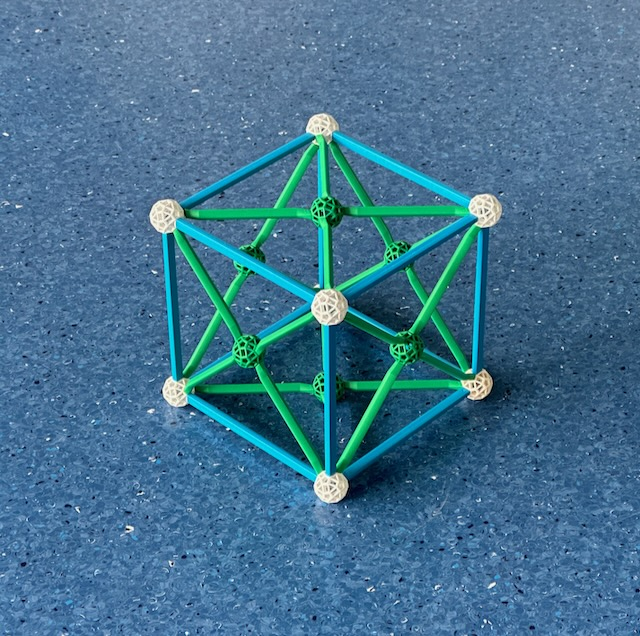Fig. 10c: The dual of the previous model, showing the facetings of the Cube, which appears outside, its vertices (in white) are the only real vertices in the model. All green balls represent false vertices, where edges intersect but don't end. Further in, with the same vertex arrangement of the Cube, is the compound of two Tetrahedra, this also appears here because it is is a self-dual compound.Fig. 11a: This is the stellation pattern of the Dodecahedron; its face is the inner pentagon. The inner pentagram is the face of the Small stellated dodecahedron. The outer pentagon is the face of the Great dodecahedron, and the outer pentagram is the face of the Great stellated dodecahedron.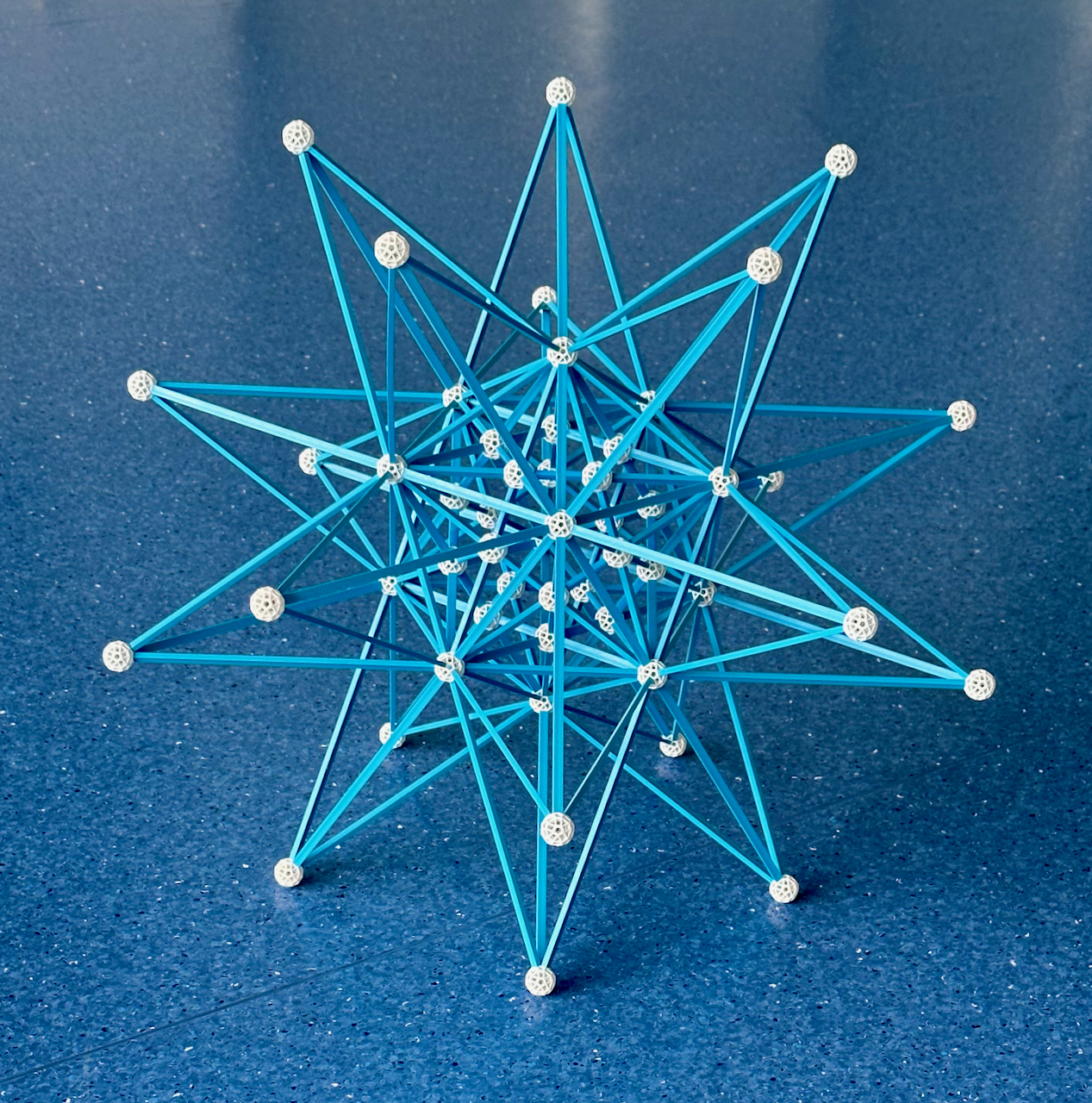Fig. 11b: Extending the 12 faces of the Dodecahedron as in Fig. 11a, we obtain the four stellations of the Dodecahedron.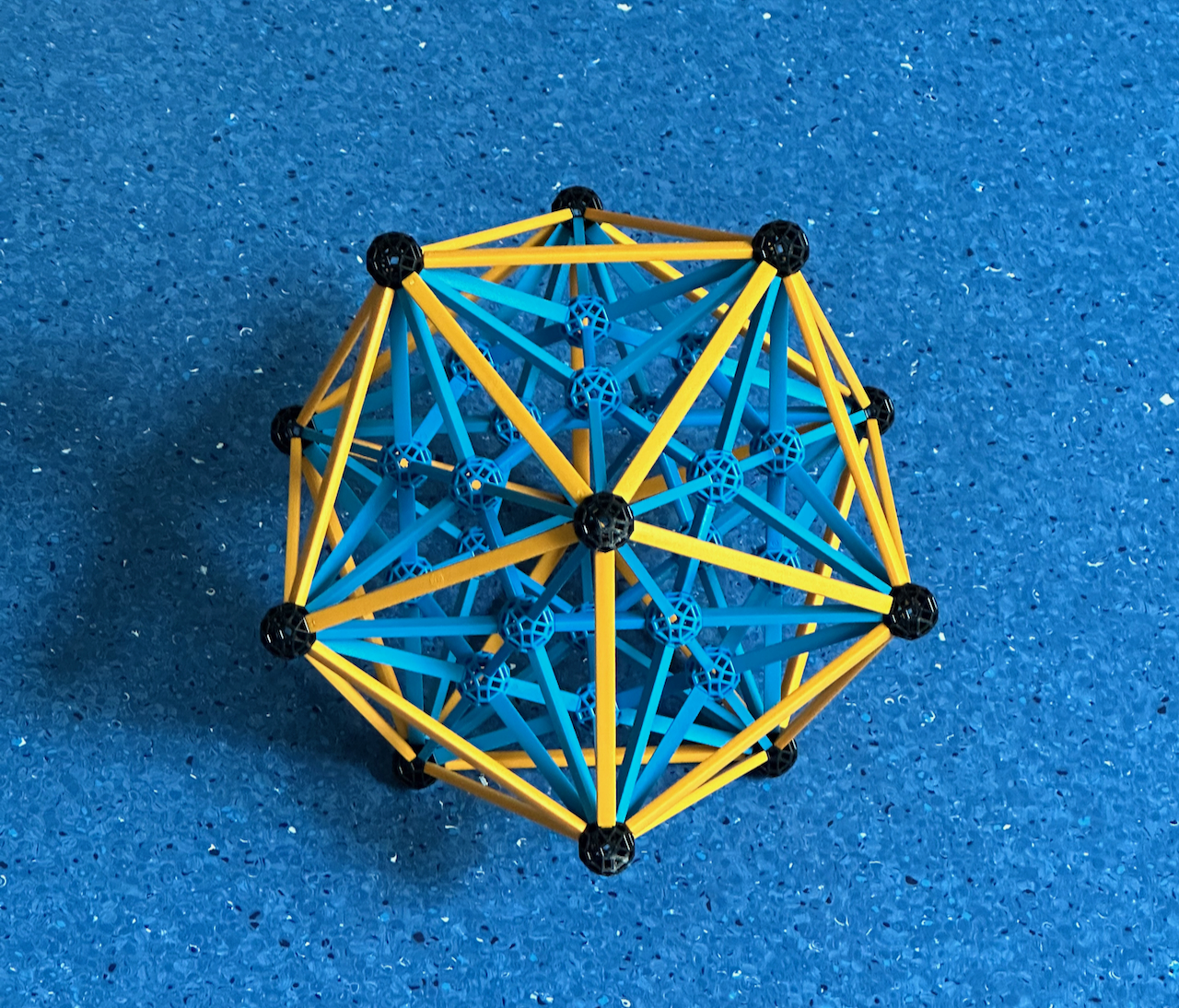Fig. 11c: In this model, the dual of the model in Fig. 11b, we represent the four facetings of the Icosahedron. The outer polyhedron is the Icosahedron, this contains all the true vertices of the model (in white). All green balls represent false vertices, where edges intersect but don't end. Further in, with the same vertex and edge arrangement but pentagonal faces, is the Great dodecahedron. Further in, with the same vertex figure of the Icosahedron, is the Small stellated dodecahedron. Finally, the innermost polyhedron, with the same edge and vertex arrangement of the Small stellated dodecahedron but with triangular faces is the Great icosahedron. The model has the same appearance as the model in 11b, except for the absence of the outer layer.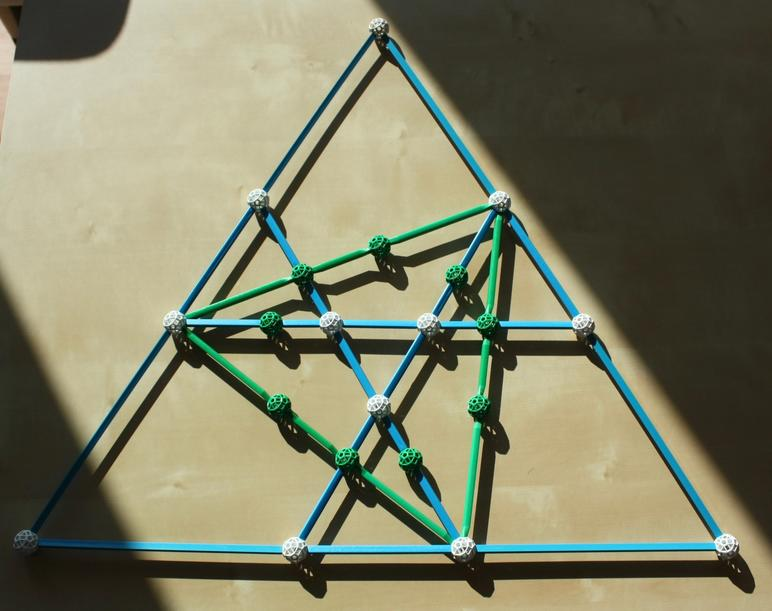Fig. 12a: An incomplete stellation diagram of the Icosahedron, representing some of the figures that can be built with a small Zometool model. The inner blue Triangle is the face of the Icosahedron, the green Triangle is a face of the compound of five Tetrahedra and the outer triangle is a face of the Great icosahedron. An intermediate figure where each face is composed of three disjointed (blue) triangles, touching the face of the Icosahedron at its vertex, is the self-dual Excavated dodecahedron (which is, therefore, another faceting of the Dodecahedron). These are only four of the 59 stellations of the Icosahedron, some others were presented already in Fig. 6.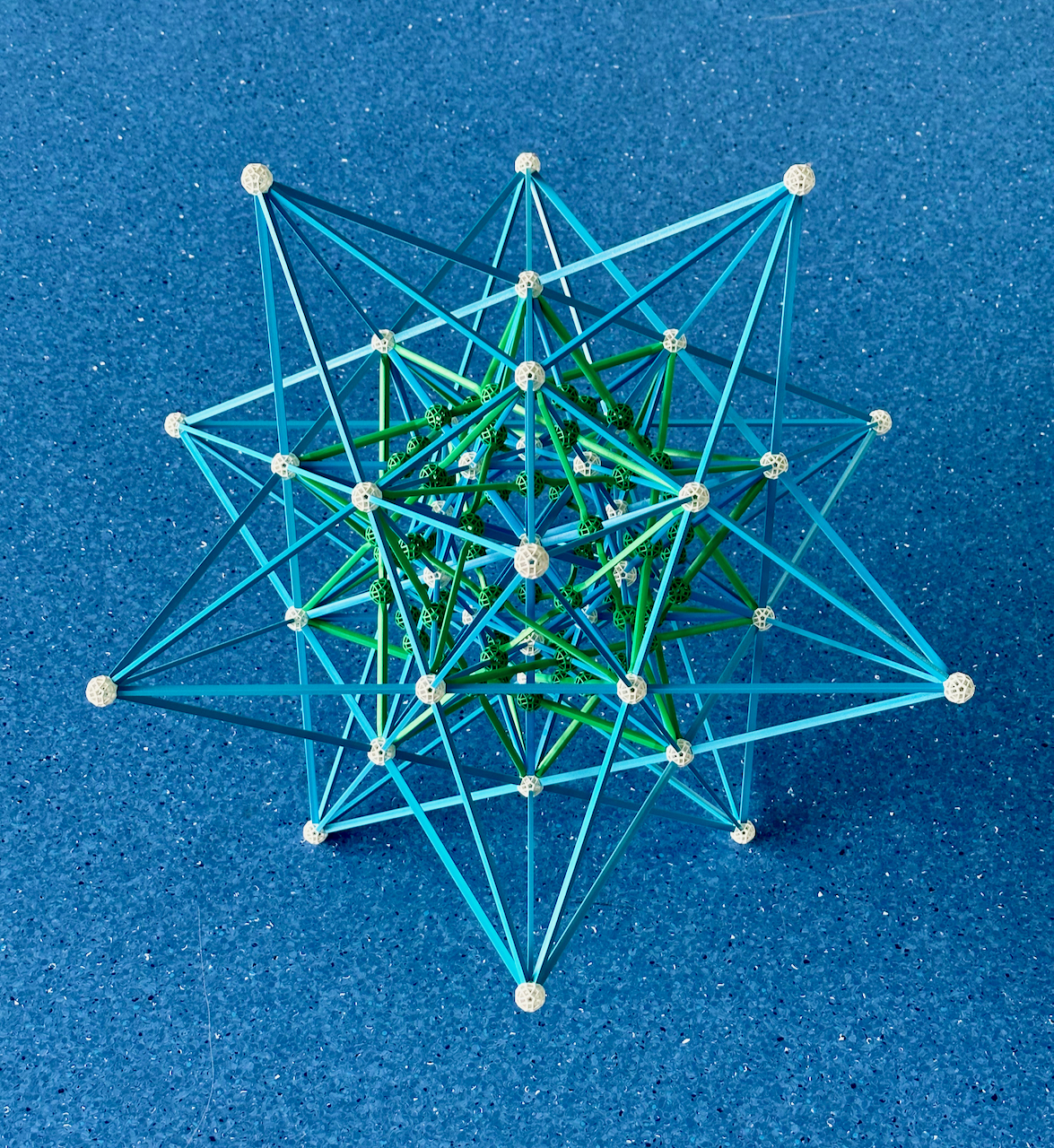Fig. 12b: Extending the 20 faces of the Icosahedron as shown above, we have the four stellations of the Icosahedron mentioned above.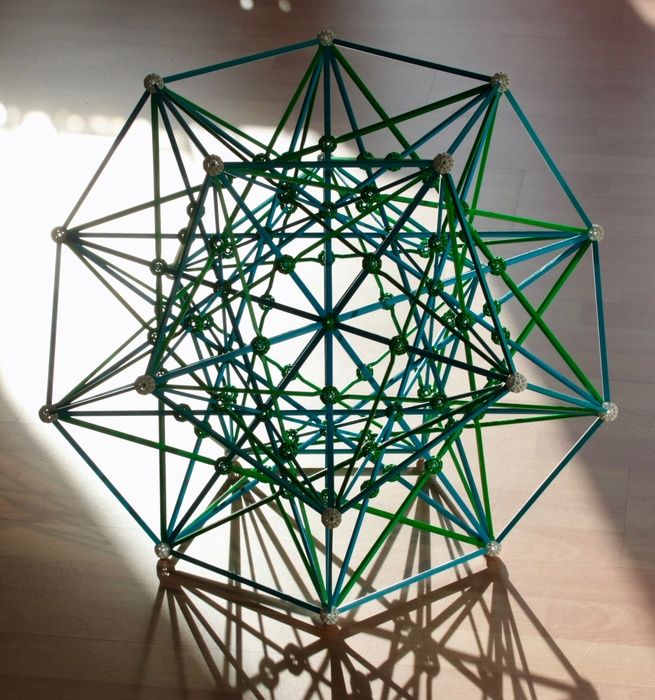Fig. 12c: In this model, the dual of the model in Fig. 12b, we represent four of the many facetings of the Dodecahedron. The outer polyhedron is the Dodecahedron, this contains all the true vertices of the model (in white). All green balls represent false vertices, where edges intersect but don't end. Further in is the Excavated dodecahedron, also present here because it is self-dual. Further in is the compound of five Tetrahedra, also self-dual. Finally, the innermost polyhedron is the Great stellated dodecahedron. The model has the same appearance as the model in 12b, except for the absence of the outer layer.

Removing the outer edges of the Dodecahedron, we obtain a model with the compound of five Tetrahedra and the Great stellated dodecahedron.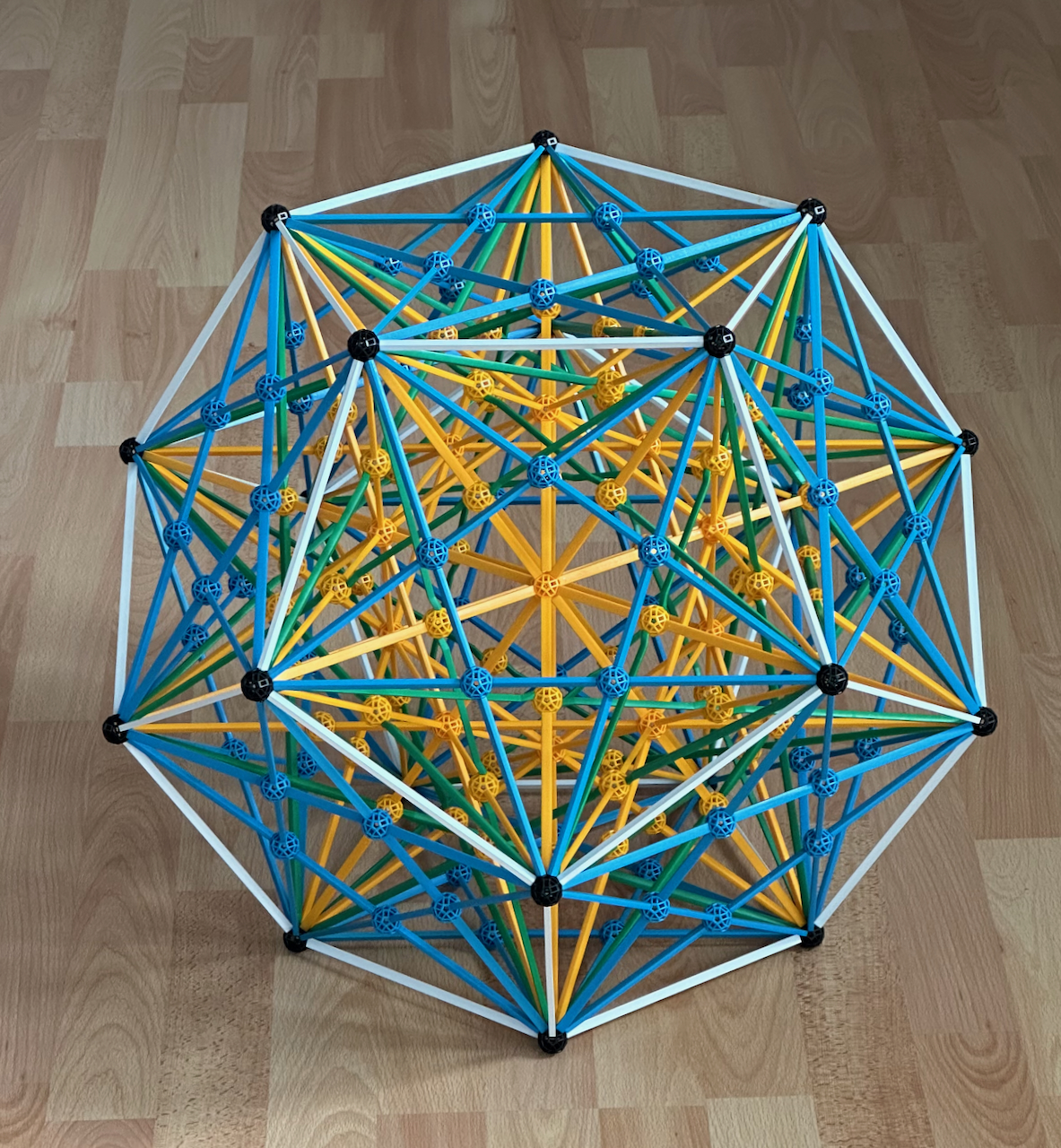Fig. 12d: One can make another very attractive model by adding the edges of another faceting of the Dodecahedron, the compound of five Cubes (which are also the edges of three other facetings of the Dodecahedron, the three ditrigonal quasi-regular polyhedra shown in Fig. 6).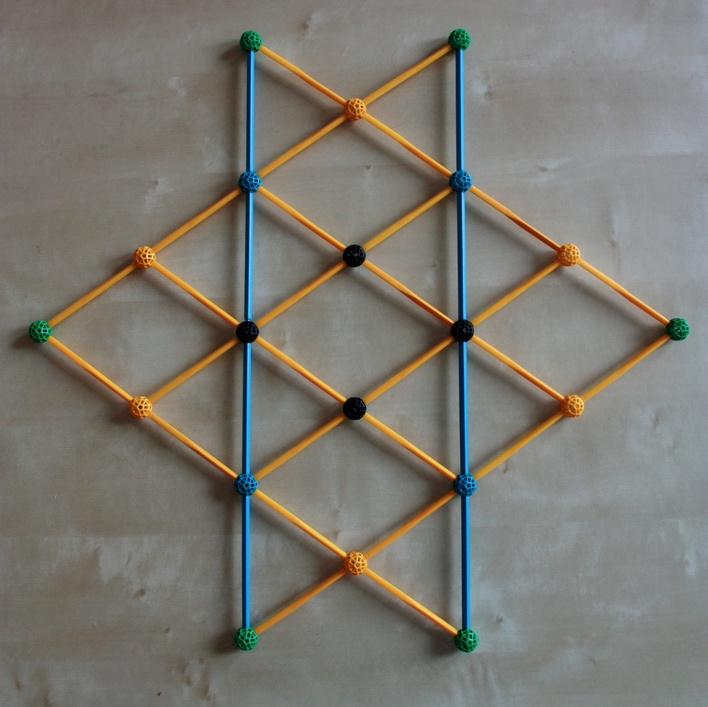Fig. 13a: The stellation diagram of the Rhombic dodecahedron built with Zometool. The inner diamond if the face of the Rhombic dodecahedron.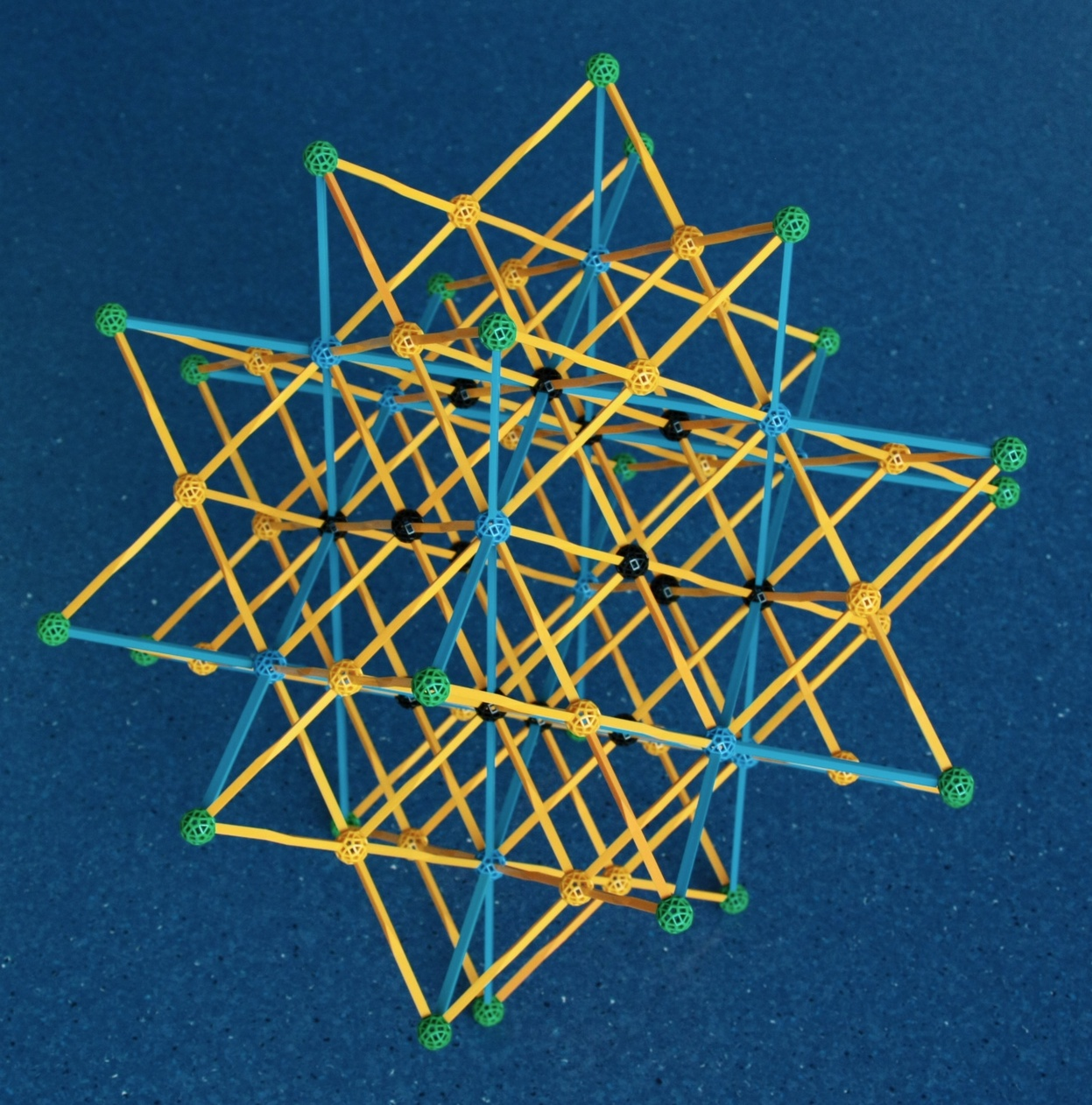Fig. 13b: The four stellations of the Rhombic dodecahedron. Each of the 12 faces of the Rhombic dodecahedron has been extended along their planes as shown above.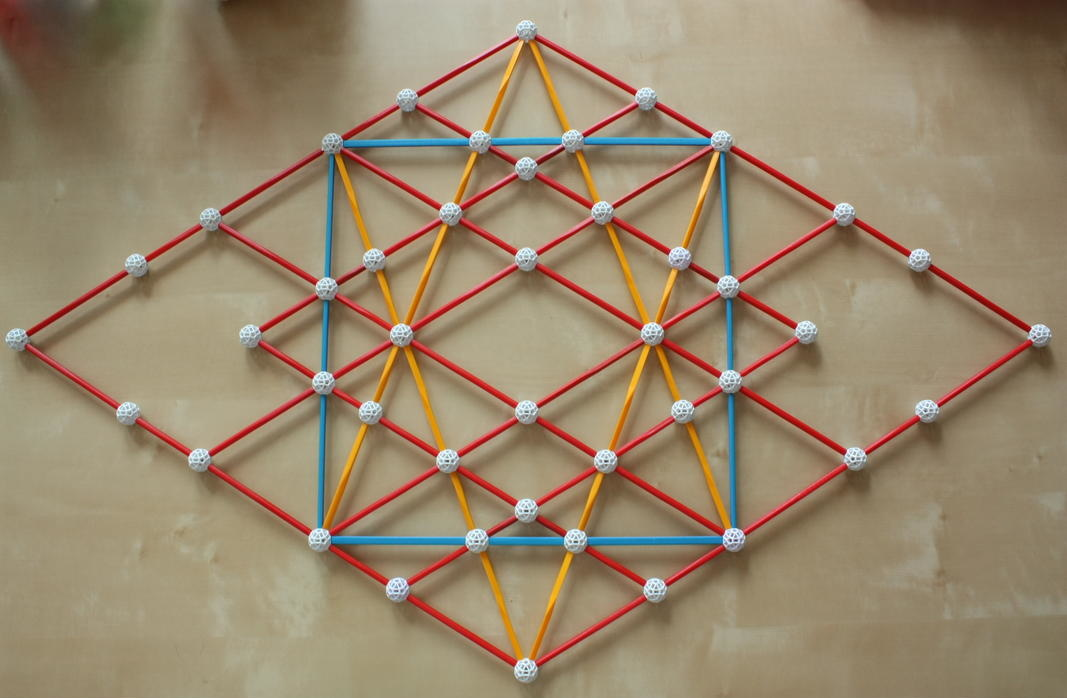Fig. 14a: The inner region of the stellation diagram of the Rhombic triacontahedron built with Zometool. The outer Rhombus is the face of the Great rhombic triacontahedron. All other struts represent intersections of its 30 rhombic faces.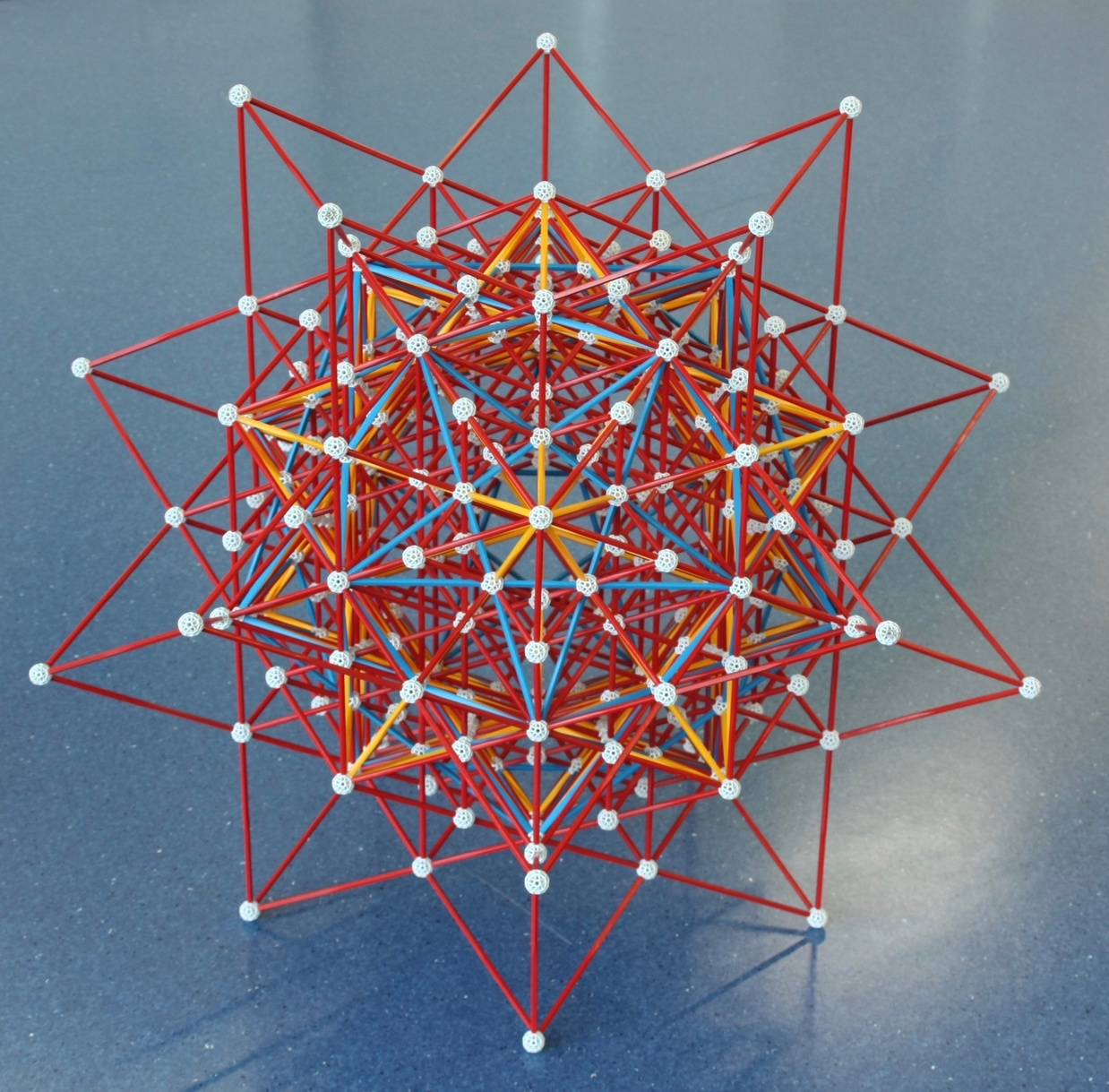Fig. 14b: If, for each facial plane we include the complete set of struts and balls as in Fig. 14a, then we obtain this model, which represents many of the stellations of the Rhombic triacontahedron up to the Great rhombic triacontahedron (the outer shape). As in Fig. 14a, every line represents the intersection of two faces of the Great rhombic triacontahedron.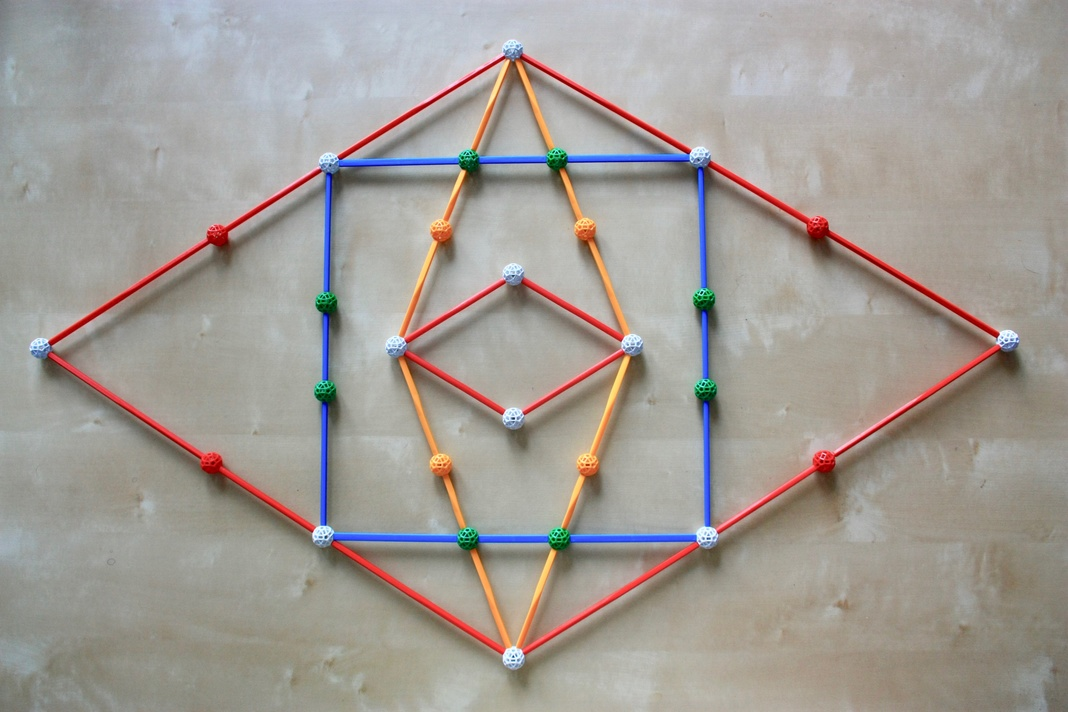Fig. 14c: Excluding most struts and connectors from the stellation diagram, we see the faces of some of the more important stellations of the Rhombic triacontahedron. The inner red Rhombus is one of the 30 faces of the Rhombic triacontahedron. The yellow Rhombus is one of the 30 faces of the Medial rhombic triacontahedron. The blue square is one of the 30 faces of the compound of 5 cubes. As in Fig. 14a, the outer Rhombus is one of the 30 faces of the Great rhombic triacontahedron.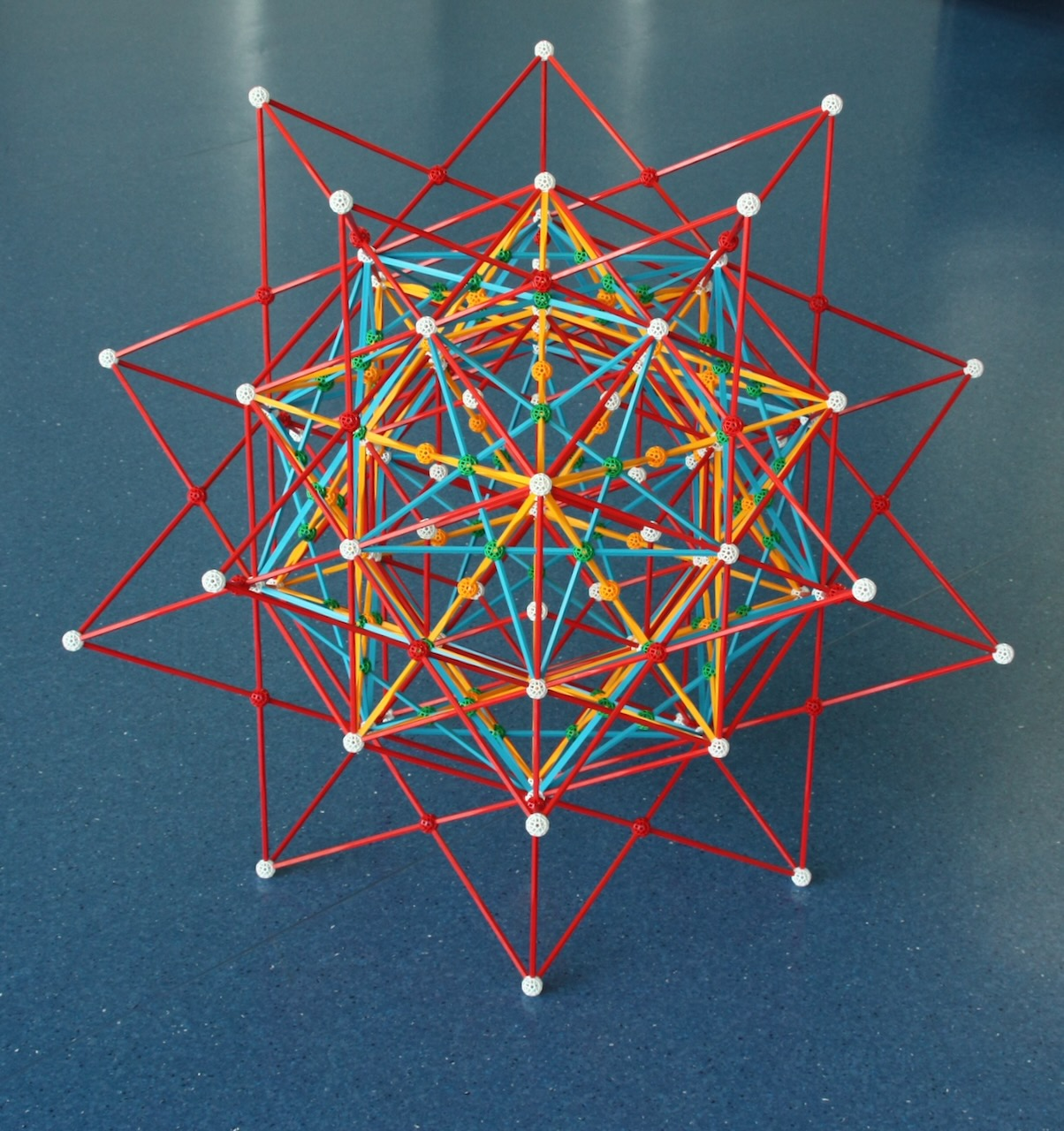Fig. 14d: Extending the 30 faces of the Rhombic triacontahedron as in Fig. 14c, we obtain this model. The four stellations can be found very easily since they are represented by different colors. This model is consistent with the ``4 stellations'' theme set by the previous models.

For more on the latter models and instructions on how to build them, see my page on the many stellations of the Rhombic triacontahedron.

## The fourth dimension: Regular and Uniform Polychora

``As for the analogous figures in four or more dimensions, we can never fully comprehend them by direct observation. In attempting to do so, however, we seem to peep though a chink in the wall of our physical limitations, into a new world of dazzling beauty."
H. S. M. Coxeter, in the preface of ``Regular Polytopes".

The generalization of polygons in 2 dimensions and polyhedra in 3 dimensions to any number of dimensions is a polytope. I am particularly interested in 4-dimensional polytopes, the polychora, which are finite regions of 4-D space bound by polyhedral 3-faces, or cells.

Among an unknown number of uniform polychora, sixteen (again!) are regular: six are convex and ten are non-convex; the latter are known as the Schläfli-Hess polychora. All have orthographic projections to 3-dimensional space that can be represented in the Zometool system, these are shown below.

Since all these models have a lot of important internal structure, there is no alternative to building them in Zometool: a paper model would hide the internal structure of the projection.

Before proceeding, a short explanation of the meaning of duality between two polychora. To a cell in a polychoron corresponds a vertex in its dual (the line from the center to the vertex is perpendicular to the 3-D plane of the cell); this vertex has a vertex figure that is the dual of the original polychoron's cell. Conversely, to each vertex in the polychoron corresponds a cell in the dual polychoron, this is the dual of the vertex figure of the original polychoron. To a face in the polychoron corresponds a perpendicular edge in its dual, which goes through the original face's center; conversely, to an edge in the polychoron corresponds a perpendicular face in its dual. Also, as in three dimensions, if polychora A and B are duals, then the dual of the stellation of A is a faceting of B. It is important to keep this in mind for understanding what follows.

Finally, a note on the names. Many 4-dimensional regular polychora have many names - one of them is known as the 120-cell, Dodecaplex, Hyperdodecahedron, Polydodecahedron, Hecatonicosachoron, Dodecacontachoron and Hecatonicosahedroid. The convention adopted here is the same adopted by Wikipedia and Coxeter's ``Regular polytopes'', which is to use the simpler names, for this example ``120-cell''. This is consistent with the names of the regular polyhedra, which refer only to the number of faces, not their type. This simple system works because the resulting names are unambiguous. Furthermore, ``120-cell'' is much easier to understand, and shorter to write, than the equivalent Greek name, ``Hecatonicosachoron''. The only exception is that for the 8-cell we will use the widely known term ``Tesseract''. This is also consistent with the polyhedral names, where the term ``Cube'' is at the same time technically precise and does not reflect the number of faces.

### Convex Regular and Uniform Polychora

For the convex regular polychora, we also display the corresponding rectified polychora. These are, among the uniform polychora, the 4-D equivalents of the quasi-regular polyhedra discussed above. As in 3 dimensions, the regular polychora and their rectified versions share the same Wythoff construction: Their vertices are reflections of one of the four vertices of the Goursat tetrahedron, the fundamental domain of their 4-D point symmetry group. This implies that for each dual pair of dual regular polychora, there are two rectified polychora. In three dimensions, for each pair of dual regular polyhedra there is only one rectified (or quasi-regular) polyhedron.

All of these polychora have orthographic projections that can be represented by Zometool models.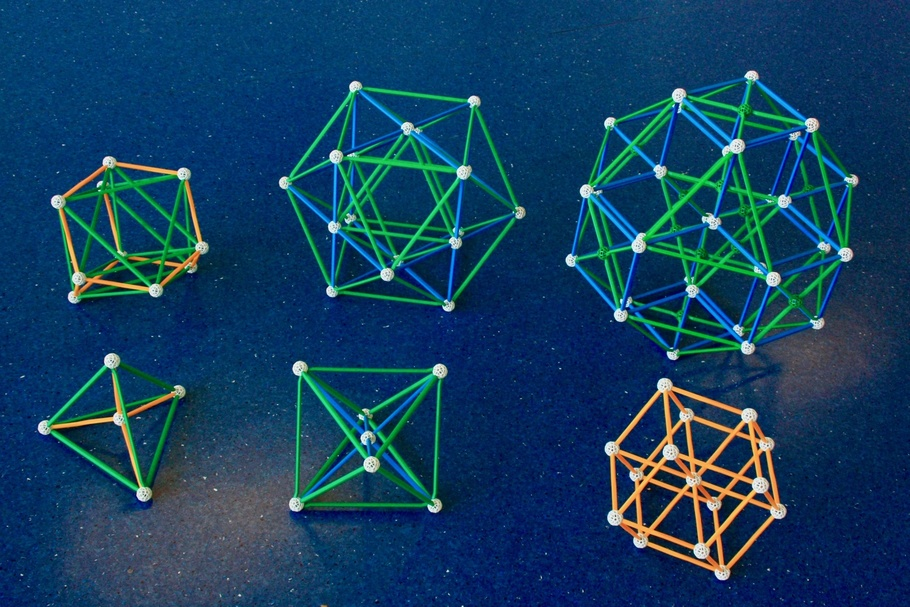Fig. 15a: Front row: Vertex-first projections of three convex regular polychora: On the left is the simplest regular polychoron, the 5-Cell, which is bound by 5 Tetrahedral cells and has 5 vertices, with Tetrahedral vertex figures. This is the 4-dimensional analogue of the Tetrahedron. In higher dimensions, the analogues are known as Simplexes, all of them self-dual. This is one of the few projections in this page where the "near" and "far" sides of the projections do not coincide.
At the center is the vertex-first projection of the 16-cell, which is bound by 16 Tetrahedral cells and has 8 vertices with Octahedral vertex figures. This the 4-D analogue of the Octahedron. The analogues in any dimension are known as Orthoplexes.
On the right is the projection of its dual, the 8-Cell, more commonly known as the Tesseract. This is bound by 8 Cubes and has 16 vertices with Tetrahedral vertex figures. This is the 4-D Hypercube.
As one might expect, Tesseracts can fill 4-D space, but more interestingly, the 16-cell can do that as well: The vertex arrangement of this 16-cell honeycomb is known as the F4 lattice. If a 3-sphere is centered in each point of this lattice, the resulting arrangement is the densest possible regular sphere packing in 4 dimensions, with kissing number 24.

In the row further back are the rectifications of these polychora (also, rectifications of the particular projections in the first row).
On the left is the cell-first projection of the Rectified 5-cell. For each of the 5 Tetrahedra of the 5-cell, this has 5 rectifications of the Tetrahedron, or Octahedra. Each vertex of the 5-cell was replaced by its vertex figure, a Tetrahedron. This is one of the 3 non-regular convex uniform polychora that has only regular cells, these are known as a Semi-regular convex polychora. In particular, this is a member of a multi-dimensional family of Semi-regular polytopes, where the facets are Simplexes and Orthoplexes, known as the k21 polytope family, where k is the dimension − 4. Thus the Rectified 5-cell is the 021 polytope. See more about this family here. This is another of the projections in this page where the "near" and "far" sides of the projections do not coincide.
On the right is tetrahedral cell-first projection of the Rectified tesseract. Each of the 8 Cubes of the Tesseract was replaced with rectifications of the Cube, Cuboctahedra. Each of the 16 vertices of the Tesseract was replaced by the Tesseract's vertex figure, Tetrahedra. This is another case of a model where the "near" and "far" sides of the projections do not coincide: this is the reason why the model displays two crossed Tetrahedra at the center (the "Stella Octangula" design).

The two models on the left have pentachoric symmetry, the other four share the hexadecachoric symmetry, but one of them (the Rectified 16-cell, below) has a higher degree of symmetry.Fig. 15b: In Fig. 15a we displayed the vertex-first projections of the 16-cell and Tesseract. Here we display, in the front row, their cell-first projections. Behind them we display the rectifications of these polychora, specifically rectifications of these projections. The projection of the rectified Tesseract (top right) is different from that shown in Fig. 15a: Instead of being centered on a Tetrahedron, it is centered on a Cuboctahedron. However, the rectification of the cell-first projection of the 16-cell is identical to the rectification of the vertex-first projection in Fig. 15a. This is because both are centered on Octahedra. There is a deeper, and rather beautiful reason for this, see section on regular polychoron compounds below.

This projection of the 16-cell is especially good for highlighting its 8 vertices separately. These represent half of the vertices of the Tesseract, its dual polytope, i.e., the 16-cell is a ``Demi-tesseract'', the 4-D Demi-hypercube (the 3-D version, the Tetrahedron is a ``Half-cube'', see the ``Stella Octangula'' model in Fig. 10). This particular projection also represents two superposed 16-cells that together have all the vertices of the Tesseract.
Note: the two models on the right are not permanently in my office.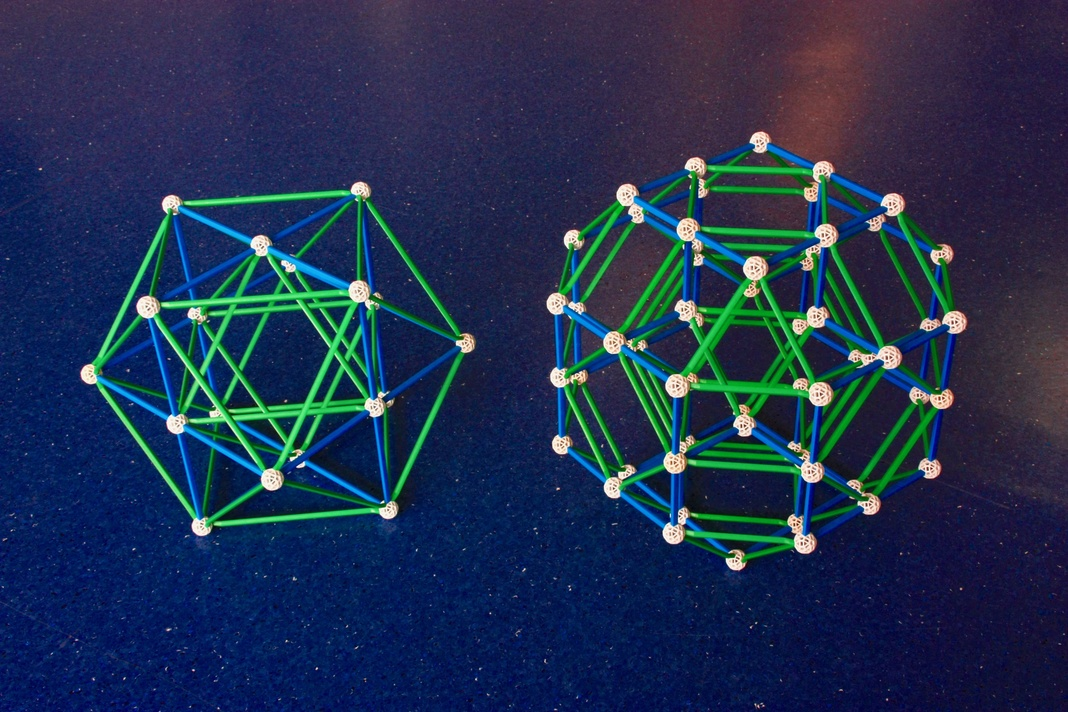Fig. 16: Left: Yet again, the Rectified 16-cell. For each of the Tetrahedral cells of the 16-cell, this has their rectifications, 16 Octahedra. The 8 vertices of the 16-cell are here replaced by the latter's vertex figures, also Octahedra. This is therefore a regular polychoron bound by 24 Octahedra in total; it is more commonly known as the 24-cell. This polychoron has many interesting characteristics: It is a self-dual polychoron with no analogue in any dimension other than 4. Its 24 vertices are those of the Tesseract and the 16-cell combined, or the combination of three 16-cells (since the 16-cell is a Demitesseract). A set of 3 16-cells with the vertices of the 24-cell is a regular polychoron compound, this is presented in Figs. 28a, b and c. Any model of the 24-cell will also represent the dual of the compound of 3 16-cells, the regular compound of three Tesseracts. For more on this see Fig. 28d.
For more projections of the 24-cell, see Figs. 27a and 27b.

The 24-cell can fill 4-D space, in what is known as the regular Icositetrachoric honeycomb. This is dual to the 16-cell honeycomb mentioned in the caption to Fig. 15a.

Right: A cuboctahedral cell-first projection of the Rectified 24-cell. For each Octahedron of the 24-cell the latter has 24 Cuboctahedra (the rectification of the Octahedron). For each of the 24 vertices of the 24-cell, the Rectified 24-cell has a Cube (the vertex figure of the 24-cell). This polychoron has 96 vertices, one for each edge of the 24-cell.

How to build: Have a look at the Eusebeia page on the Rectified 24-cell. For this particular model, see the part on the "Cantellated 16-cell", for the pyritohedric projection, see the first part of the page.
Both polychora have icositetrachoric symmetry.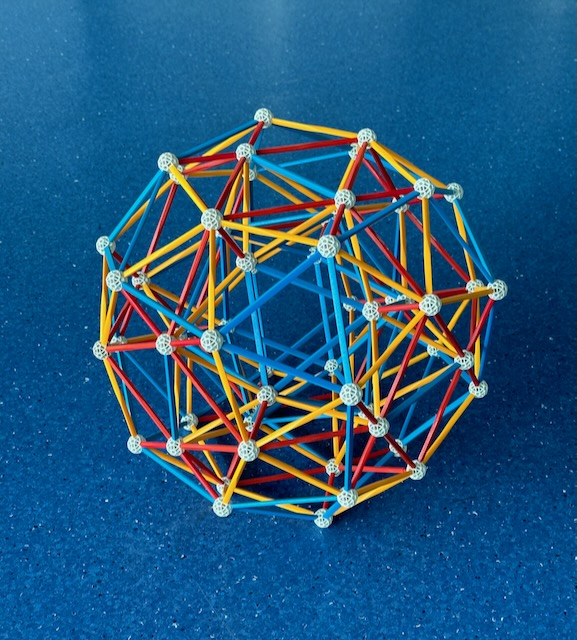Fig. 17: This is an Icosahedral cell-first projection of a convex uniform polychoron, the Snub 24-cell. This can be built from the Rectified 24-cell in Fig. 16 by diagonally bisecting all squares. If the diagonals are chosen correctly, then each of the 12 vertices of the Cuboctahedron now has 5 edges converging on it, i.e., it has been transformed into an irregular Icosahedron. The Cubes have been transformed into sets of 5 Tetrahedra, with only the central one being regular. Then we shorten the diagonals of these former squares in order to make two regular triangles. This generates one regular Icosahedron for each of the previous 24 Cuboctahedra, and five regular Tetrahedra for each of the 24 previous Cubes, making a total of 120 Tetrahedra. This operation preserved the number of vertices, which is still 96. Because all cells are regular, this is also a semi-regular convex polychoron.

How to build: Have a look at the Eusebeia page on the Snub 24-cell. Also recommended is David Richter's page on the 24-cell and its snub.

Unlike the polychoron models shown above, this polychoron is neither regular nor a rectification, i.e., its Wythoff construction is very different from most polytopes in this page. The vertices and edges of this model are a subset of those of the model of the 600-cell just below, i.e., this is a diminished 600-cell.
Picture by Jason Wu.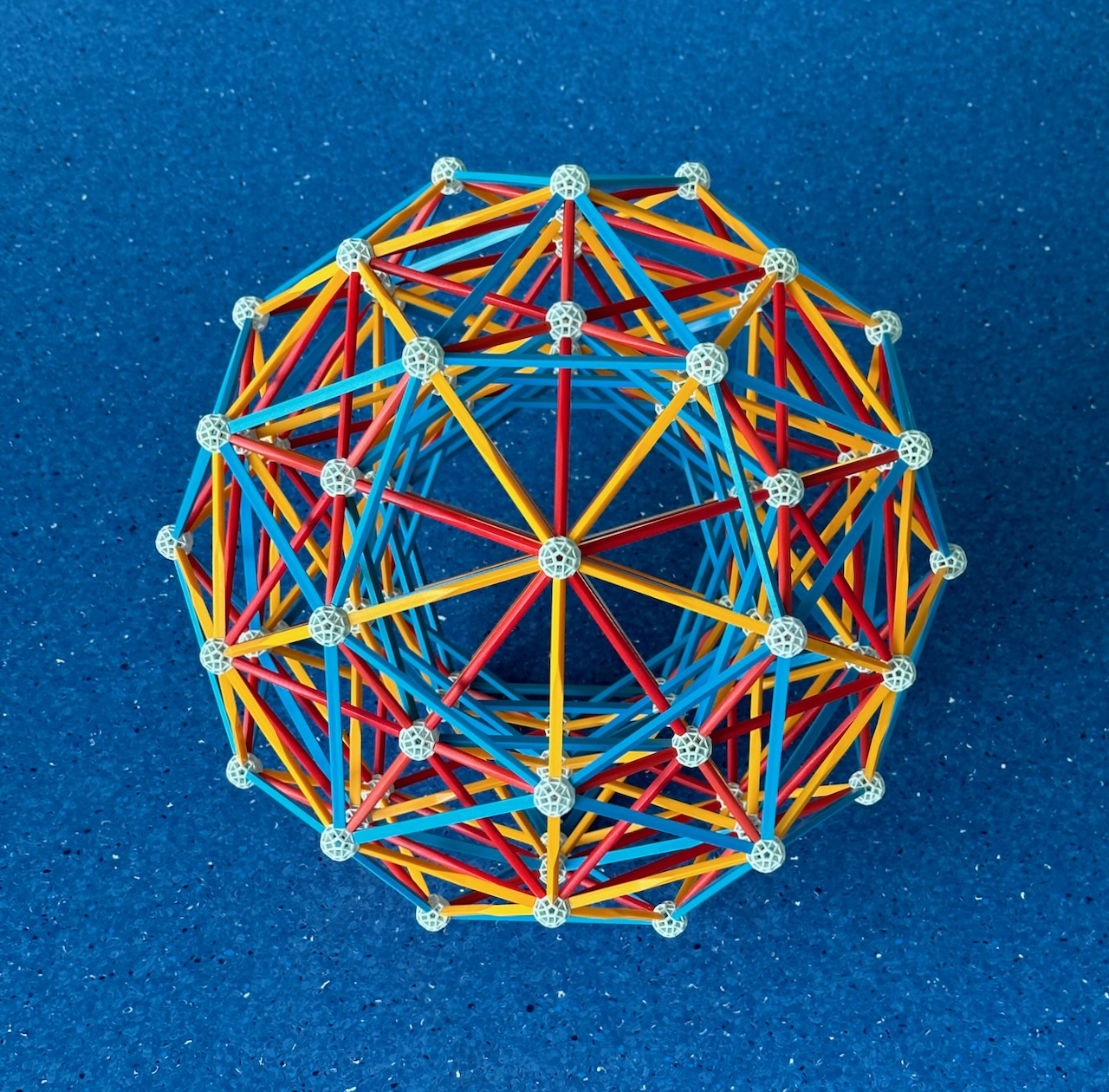Fig. 18: This is the vertex-first projection of a regular polychoron known as the 600-cell. This is a 4-D analogue of the Icosahedron.
This polychoron can be obtained from the Snub 24-cell in Fig. 17 by adding on ``top'' of each of the latter's 24 Icosahedra a 4-D ``Pyramid'' consisting of an Icosahedral ``base'' and 20 regular Tetrahedra as ``sides''. The 24 apices of these Pyramids have the same arrangement as the vertices of a 24-cell. Together with the previous 96 vertices of the Snub 24-cell, they add to a total of 120 vertices. The 120 Tetrahedra of the Snub 24-cell are now joined by the 24 × 20 Tetrahedra = 480 Tetrahedra of the new 4-D ``Pyramids'', making a total of 600 Tetrahedral cells. The apices of the new ``Pyramids'' have Icosahedral vertex figures, this is true for all other vertices as well because the 600-cell is regular.

How to build: see instructions in David Richter's list of Zometool projects.
Picture by Jason Wu.

The high degree of symmetry of the 600-cell implies that there is nothing special about the new 24 vertices added to the Snub 24-cell to form the 600-cell. Just as the latter have the same arrangement as the vertices of the 24-cell, the previous 96 vertices can be decomposed into 4 groups of 24 vertices, each also with the same arrangement as the vertices of a 24-cell. This means that we can build a set of five 24-cells with the same vertex arrangement of the 600-cell. This is a self-dual regular polychoron compound and is shown in Figs. 29a, b and c. Furthermore, as mentioned in the caption of Fig. 16, each 24-cell can be replaced by a set of 3 16-cells leaving the vertices unchanged. Doing this for the compound of 5 24-cells results in a regular compound of 15 16-cells, which will have the same vertices of the 600-cell. A model of this is shown in Figs. 30a and b.
Another interesting aspect of this model: the outlines of the Icosahedra that were in the Snub 24-cell are still visible. But apart from those, you will also be able to see Dodecahedra and Icosidodecahedra in the model. Studying it further, you will also see many flattened versions of those polyhedra. Studying those will make it easier to understand and build some of the models that come later.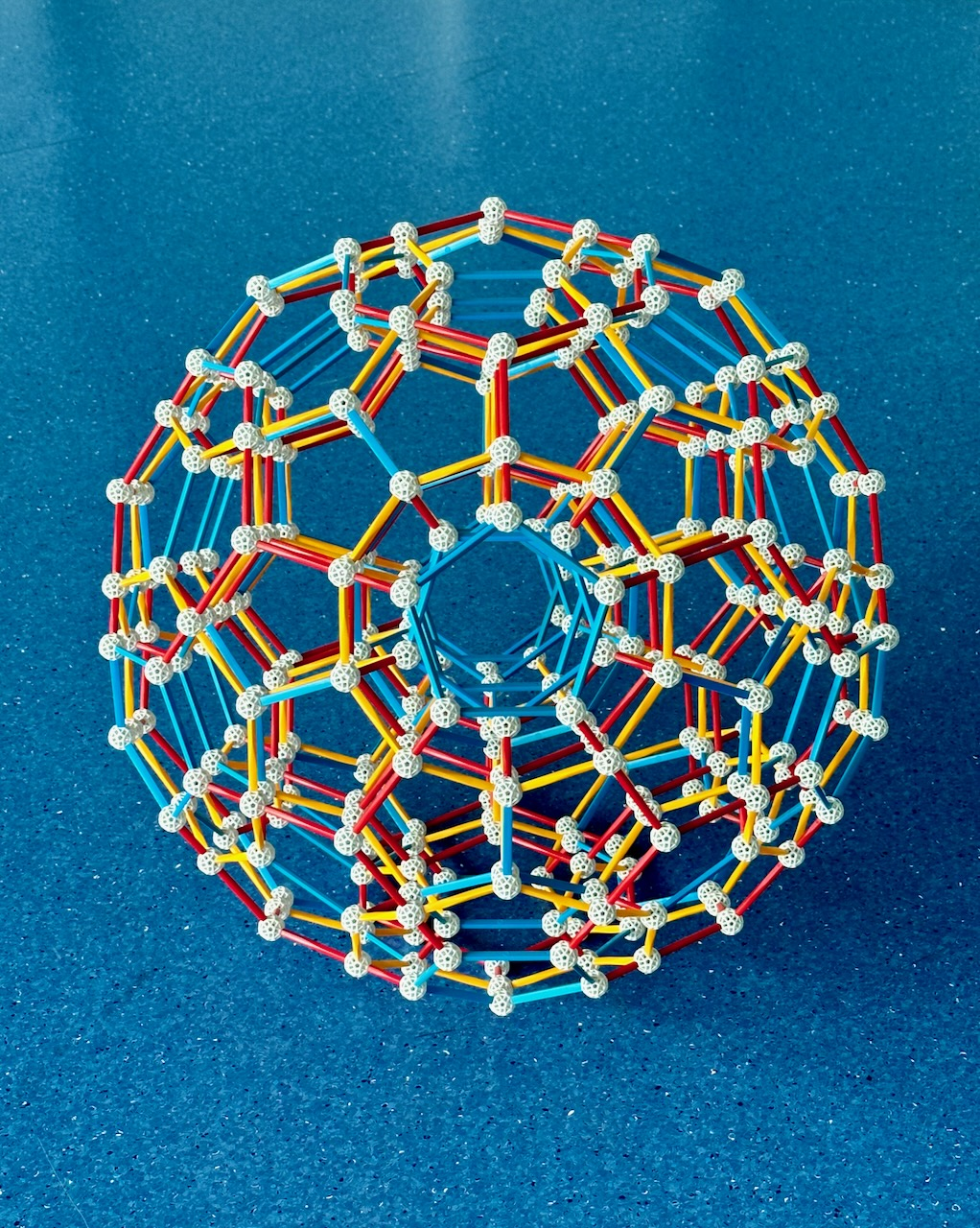Fig. 19: This is the cell-first projection of the dual of the 600-cell, the 120-cell.
This regular polychoron is bound by 120 Dodecahedra, it has 600 vertices with Tetrahedral vertex figures; this is a 4-D analogue of the Dodecahedron.
Picture taken by Jason Wu.

How to build: see instructions here. The model in the figure is larger than the set sold by Zometool as the hyperdo by φ, the famous golden ratio, φ.

This ends the list of the regular convex polychora.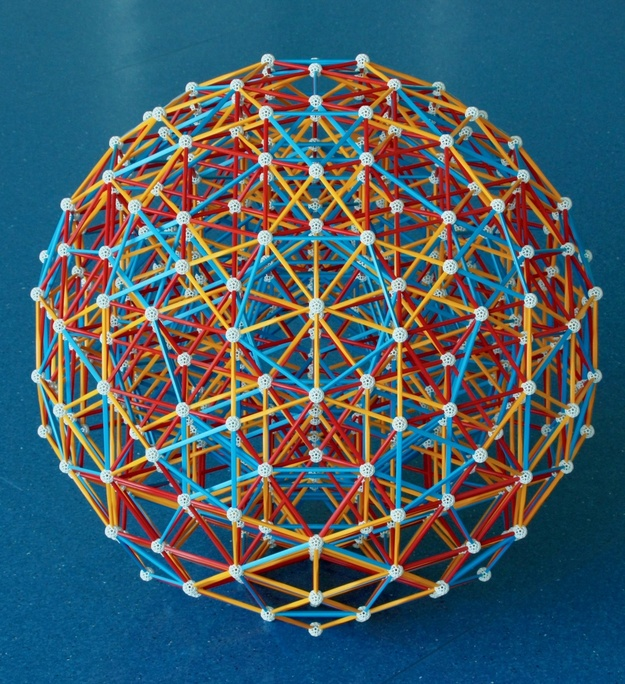Fig. 20: The icosahedral cell-first projection of the Rectified 600-cell. The 600 Tetrahedra of the 600-cell are here replaced by their rectifications, which are Octahedra. In the position of each of the 120 vertices of the 600-cell 120 Icosahedra (the vertex figure of the 600-cell) appeared. Because all cells are regular, this is the last of the three semi-regular convex polychora.

How to build: After studying the perspective-flattened Icosahedra in the model of the 600-cell, have a look at the Eusebeia page on the Rectified 600 cell. Also recommended is David Richter's page on the rectified 600-cell.

If you pay close attention to the model, you will be able to see many Archimedean solids in blue, starting with Icosidodecahedra. Studying the model further, you will be able to see many flattened versions of those. This is no coincidence: the Rectified 600-cell can be faceted to form 14 non-convex polychora with the same edge arrangement. One of them is the Rectified icosahedral 120-cell, which has Great dodecahedra and Icosidodecahedra as cells. More about the Icosahedral 120-cell below.

This model has been disassembled.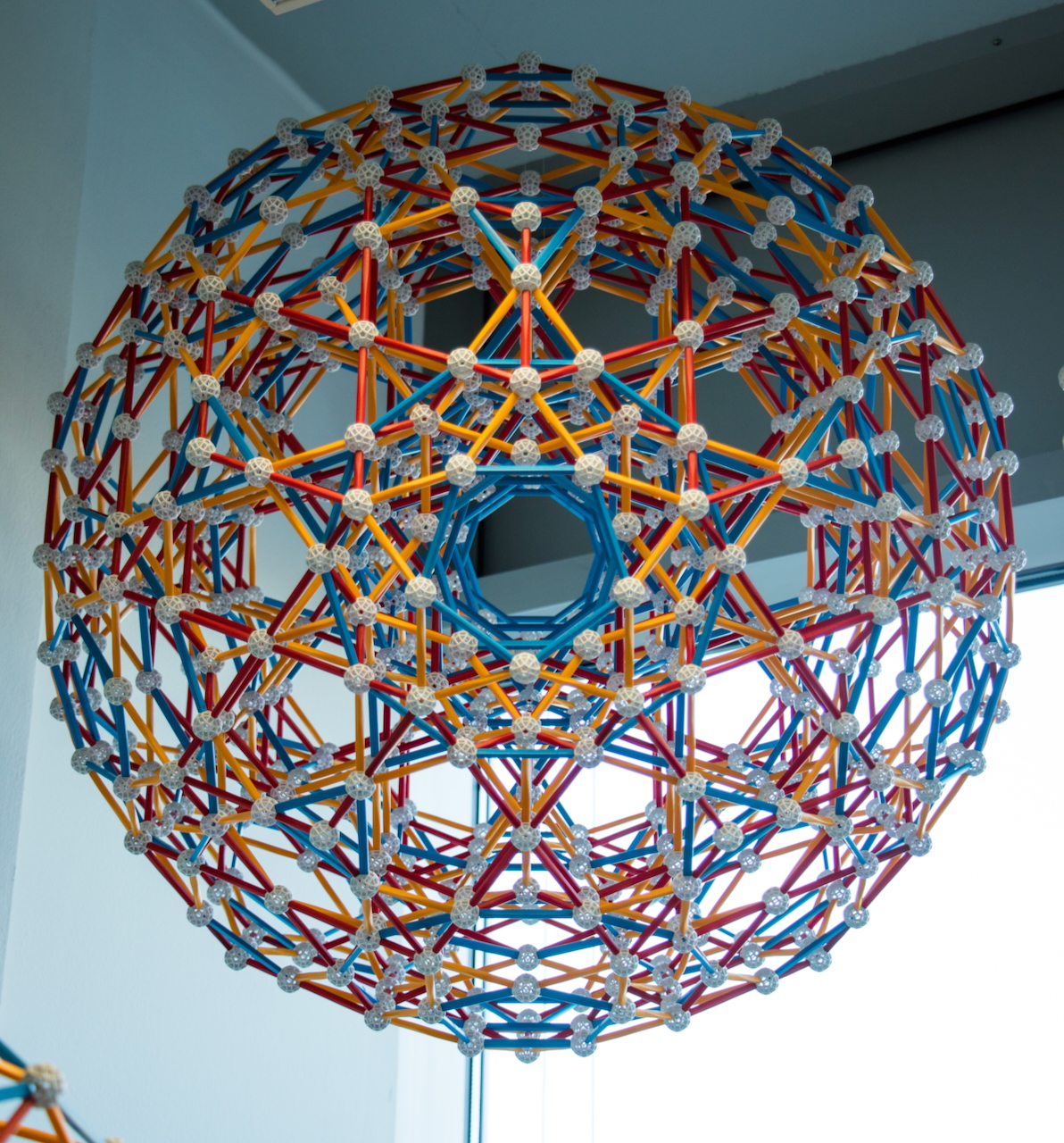Fig. 21: The icosidodecahedral cell-first projection of the Rectified 120-cell. The Dodecahedra of the 120-cell are here replaced by their rectifications, Icosidodecahedra. In the position of each of the 600 vertices of the 120-cell, 600 Tetrahedra (the vertex figure of the 120-cell) appeared.

How to build: Study the perspective-flattened Dodecahedra in the model of the 120-cell. Make models of Icosidodecahedra with similar flattening. Then have a look at the Eusebeia page on the Rectified 120 cell.
Picture taken by Jason Wu. This model has been disassembled.

The last 4 polychora have hexacosichoric symmetry.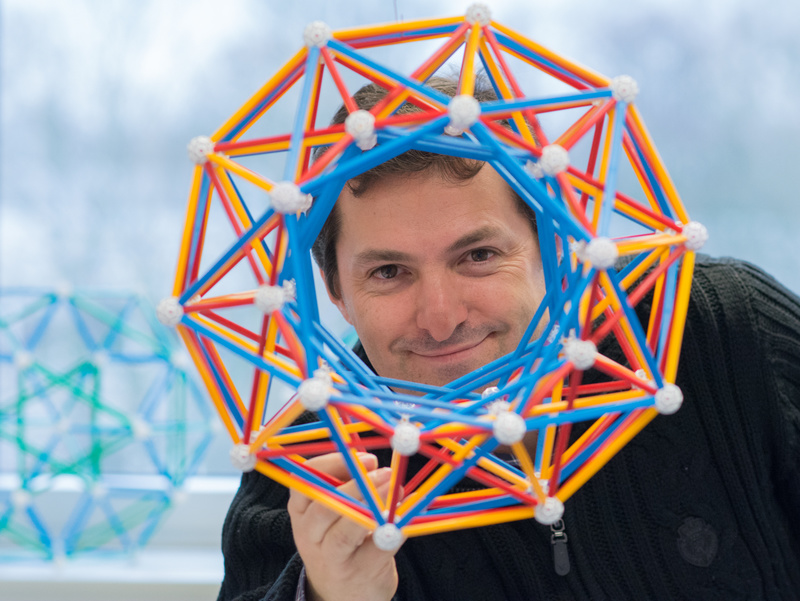Fig. 22: Another diminished 600-cell, the Grand antiprism. This was the 64th and last uniform convex polychoron, discovered in 1964 by John H. Conway and Michael Guy, who also proved that this is really the last one.

This is an anomalous uniform polychoron in the sense that, unlike all other polytopes discussed above, it has no Wythoffian construction.

Its structure also helps understanding the structure of the 600-cell, in particular the fact that the latter can be decomposed in two disjoint perpendicular rings of 150 Tetrahedra each, separated by a Clifford torus of 100 Tetrahedra with the two sets of 100 Tetrahedra that border that torus on each side. In the Grand Antiprism, both tetrahedral rings have been removed and replaced with rings of 10 Pentagonal Antiprisms; in this model we're looking through one of these rings.

How to build: Study the model of the 600-cell in detail, then have a look at the Eusebeia page on the Grand antiprism.
Picture by Aris Noutsos. Linked picture by Jason Wu.

It is important, before finishing, to note that although the models above include all the regular and ``semi-regular'' convex polychora, it includes only a small number of the 64 convex uniform polychora. Some examples I have not built are in David Richter's list of convex uniform polytopes with pentachoric symmetry and his list of convex uniform polytopes with hexacosichoric symmetry. Many of the models based on the symmetry of the Tesseract and 24-cell cannot be built in Zometool because they require the construction of regular Octagons - which are forbidden in the Zometool system.

### Non-convex Regular Polychora

We now move to the ten regular non-convex polychora, the Schläfli-Hess polychora. Nine of them can be described as stellations of the 120-cell, and one of them as a stellation of the 600-cell. The dual of each Schläfli-Hess polychoron is also a Schläfli-Hess polychoron, this implies, by the principle of duality, that nine of them are facetings of the 600-cell and one of them is a faceting of the 120-cell. For that reason, they all share the hexacosichoric symmetry of the 120-cell, 600-cell and their rectifications shown above.

These polychora are described in detail in the Wikipedia link we include for each polychoron. The geometric relations between the 120-cell, 600-cell and the 10 Schläfli-Hess polychora, together with some basic properties, are described in Figures 26a, b and c below. In Fig. 26c, we can see that three of them - the Icosahedral 120-cell (the cells are the Icosahedra mentioned in the caption of Fig. 18), the Grand 120-cell (the cells are the Dodecahedra mentioned in the caption of Fig. 18) and the Great 120-cell (the cells are Great dodecahedra) - share the same edge arrangement of the 600-cell. This makes them analogues of the Great dodecahedron, which has the edge arrangement of the Icosahedron. The Great 120-cell is a particularly good example of this, since it has Great dodecahedra as cells; the latter's edges are those of the Icosahedra mentioned in the caption of Fig. 18.

Thus the Zometool model of the 600-cell shown in Fig. 18 also represents orthographic projections of the edge arrangements these 3 Schläfli-Hess polychora!

Below are three models that represent the 7 remaining Schläfli-Hess polychora: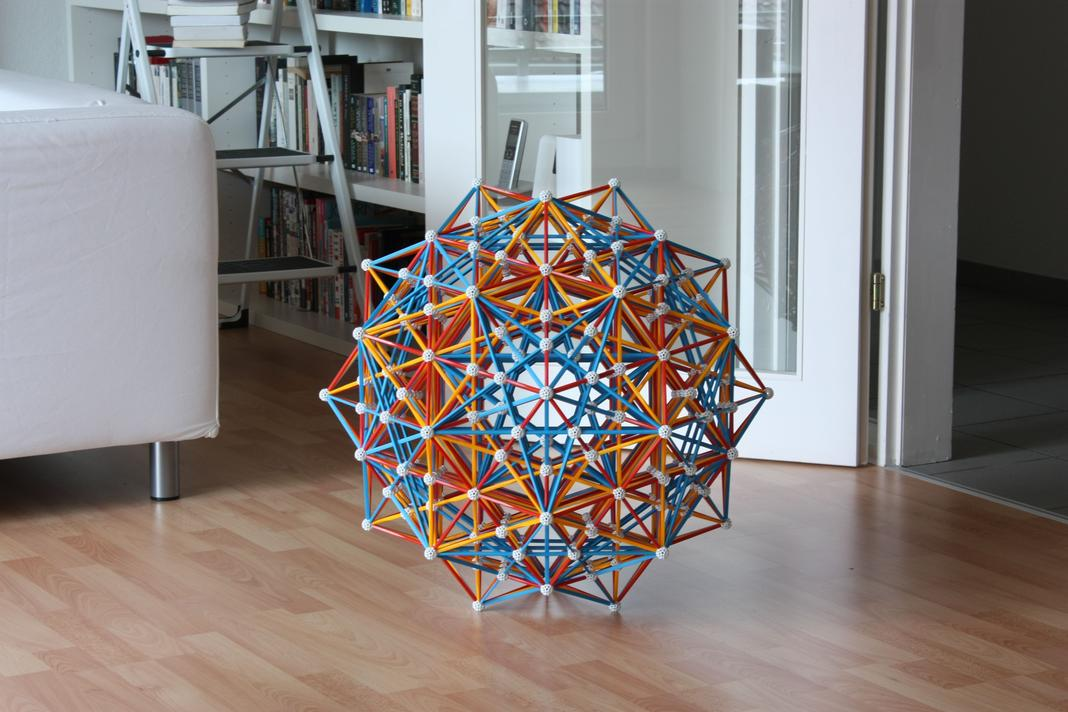Fig. 23a: This model represents the vertex-first projection of two other regular Schläfli-Hess polychora which share the same edge arrangement, the Small stellated 120-cell and the Great grand 120-cell.

How to build: see instructions in David Richter's dedicated page.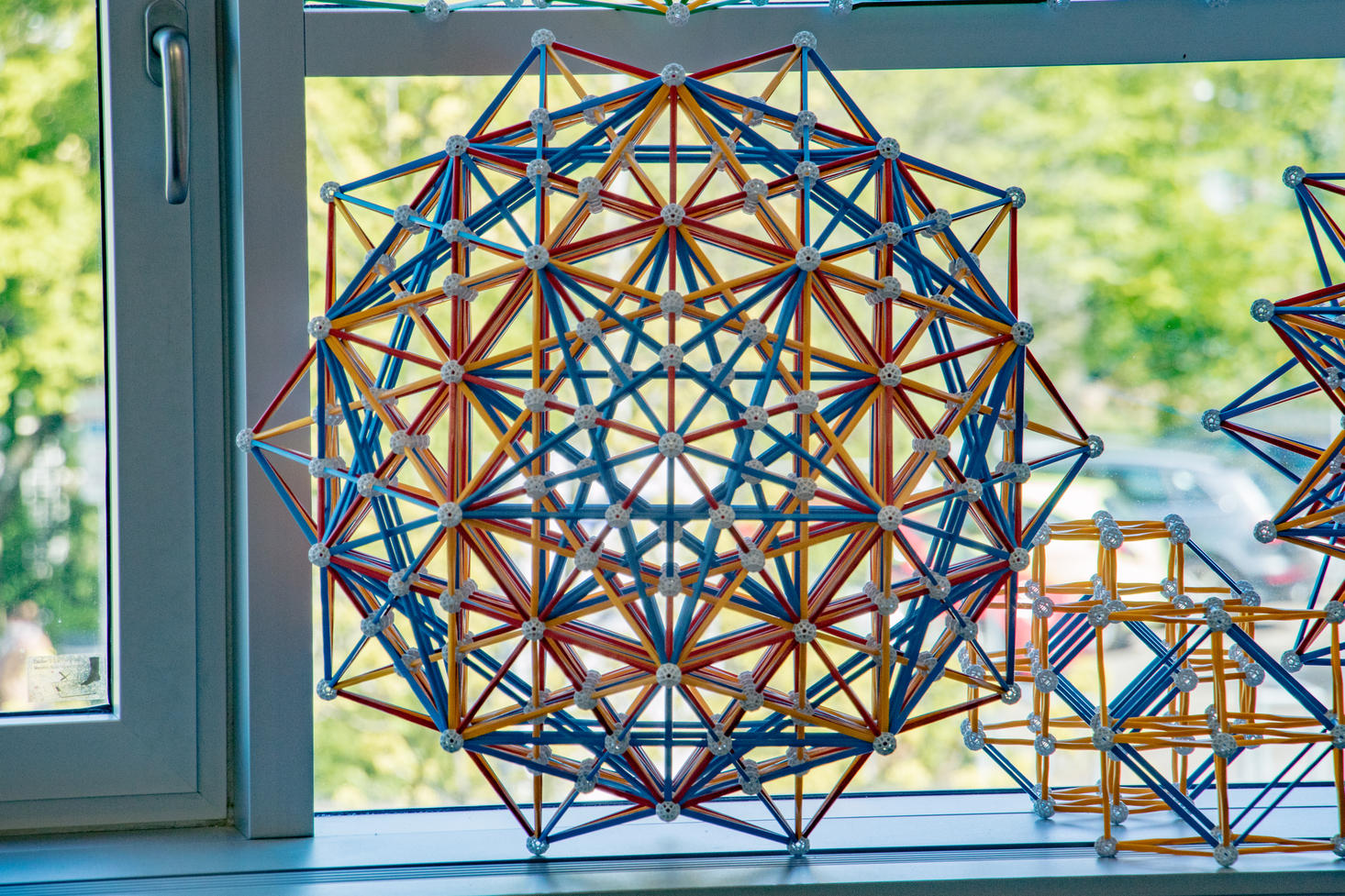Fig. 23b: This is how it looks like in my office. Picture by Jason Wu.

As shown in the caption to Fig. 23c (later in this page), this model also represents one of the two regular compounds of 25 24-cells and one of the two regular compounds of 75 Tesseracts.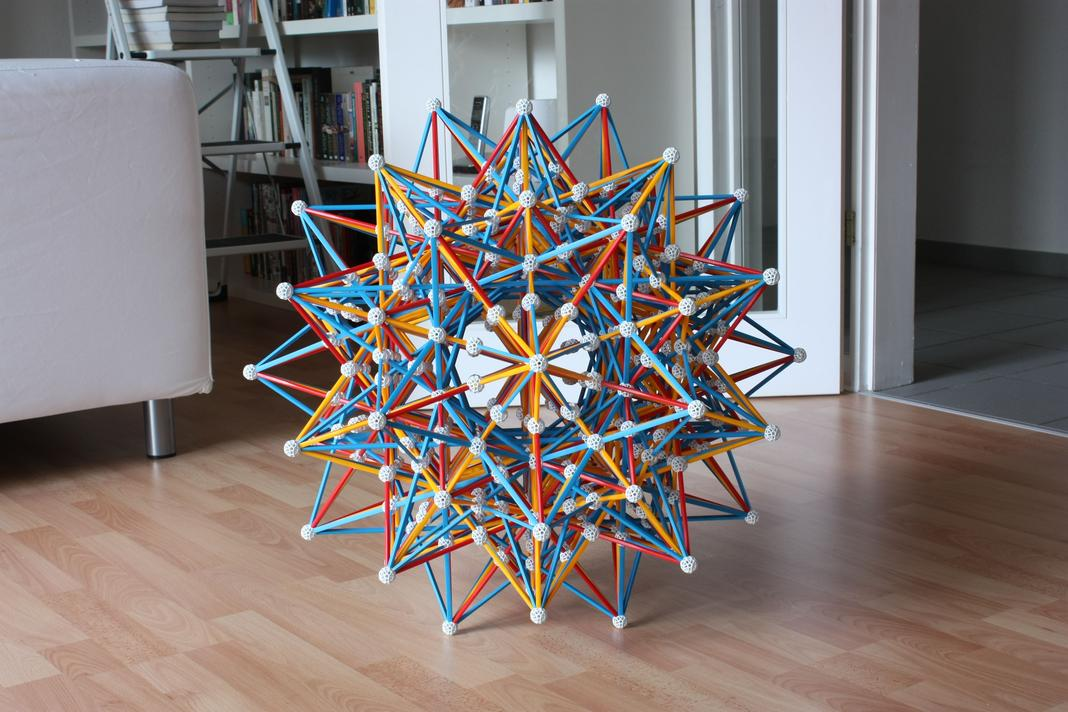Fig. 24a: This model represents the vertex-first projections of four Schläfli-Hess polychora that share the same edge arrangement: The Grand 600-cell, the Great stellated 120-cell, the Grand stellated 120-cell and the Great icosahedral 120-cell.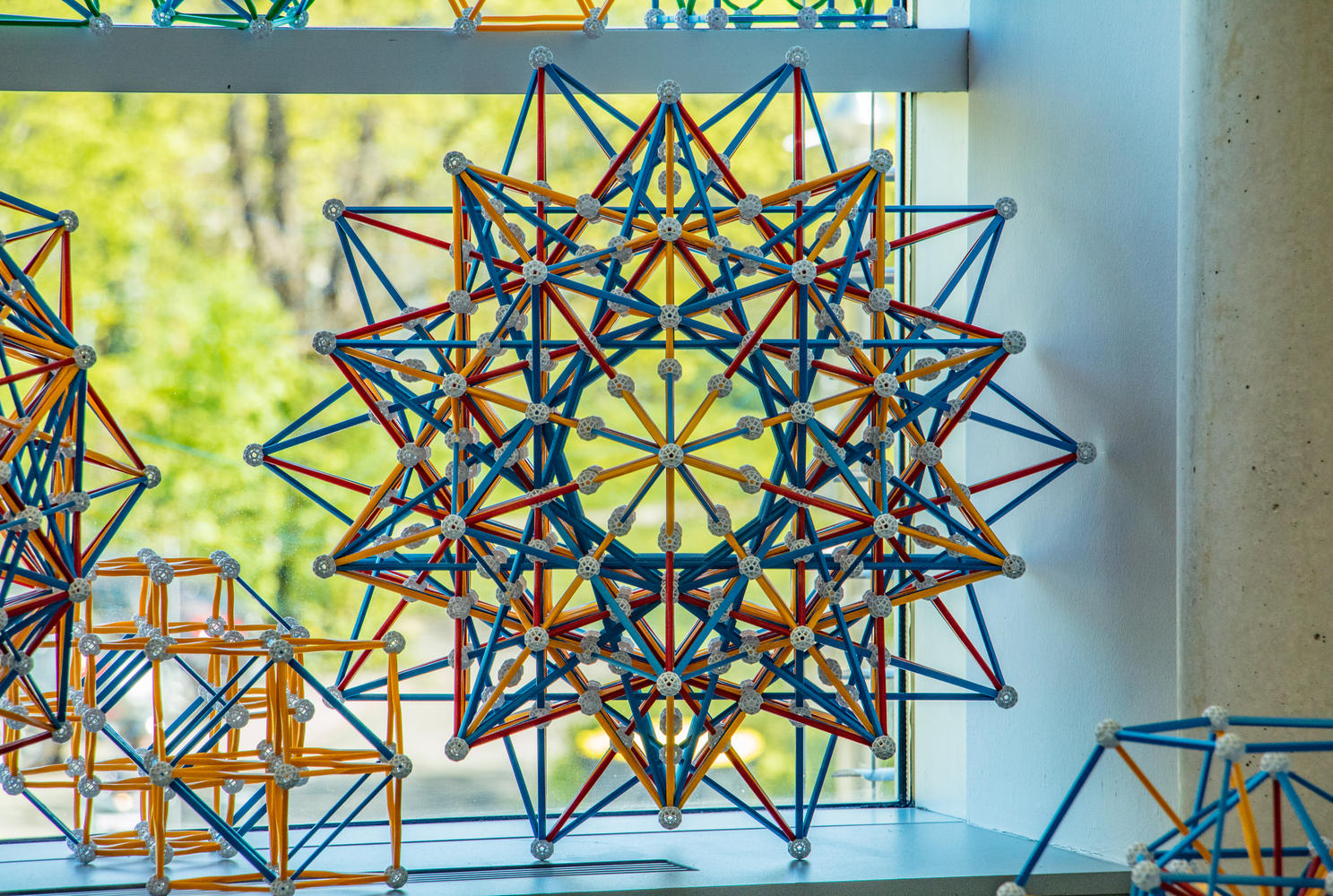Fig. 24b: This is how it looks like in my office. Picture by Jason Wu.

How to build: Make a model of the 600-cell, but extend all internal edges: there can be no broken lines. Then build a new, outer set of vertices of the 600-cell, but increased in side by the Golden ratio, (only a few of these will suffice). For each new outer vertex, see what edges of the internal model point towards it, then extend those edges to the outer vertex. Do this for a set of neighboring vertices, which will allow you to work out the intersections of those edges. Once this is done, apply the same pattern to all outer vertices using icosahedral symmetry, while removing all the edges of the outer 600-cell that were holding the outer vertices in place. For the justification of this method of construction, see the end of the caption of Fig. 26c.
Note that, as in the previous model, there are several intersecting blue edges. Because I wanted to make this a permanent addition to my office, I cut the necessary number of blue struts with pliers.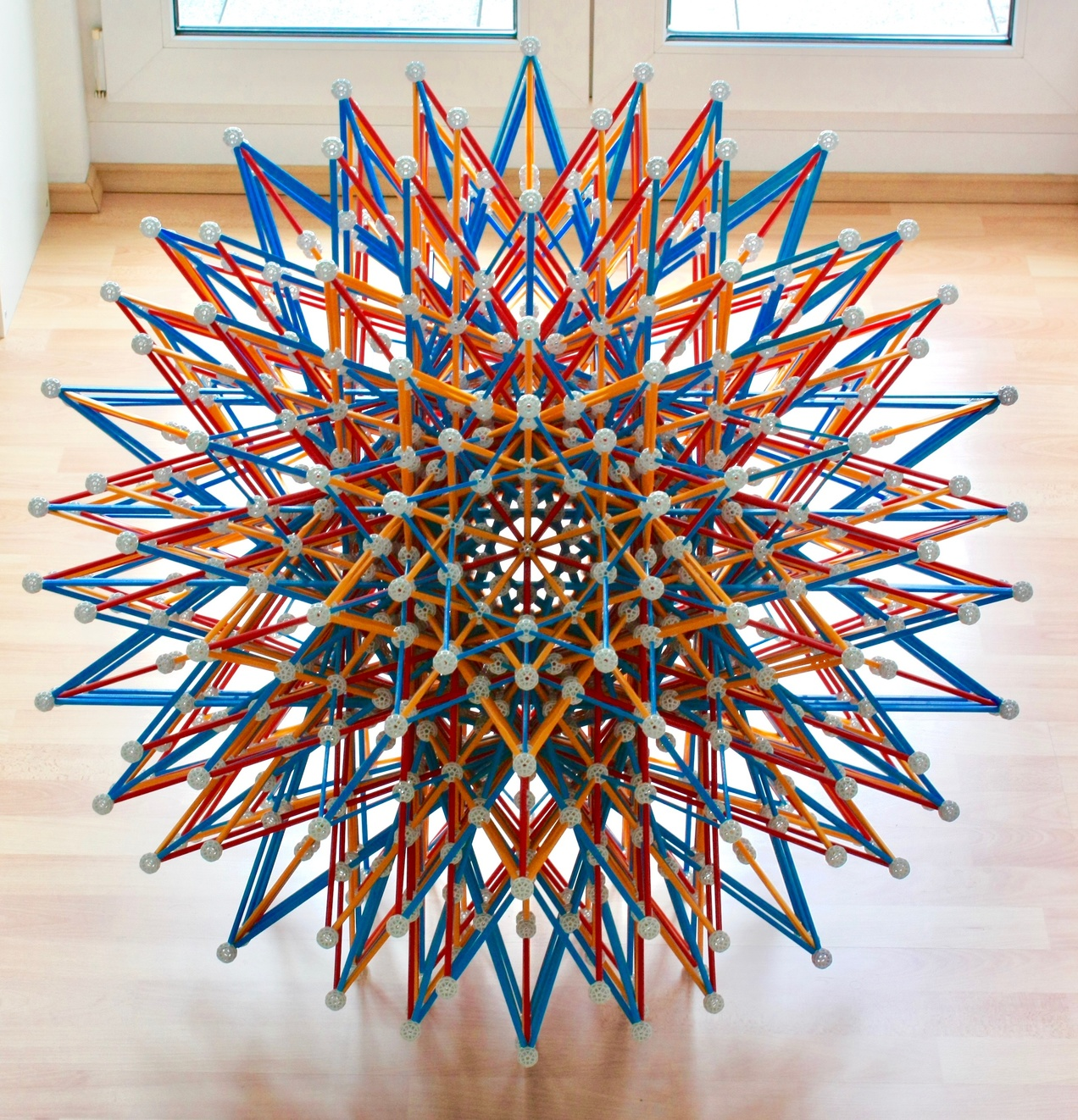Fig. 25a: The last of the Schläfli-Hess polychora, the most complex of the 16 regular polychora, the final stellation of the 120-cell: The Great grand stellated 120-cell. Here it is seen from a 5-fold symmetry axis.Fig. 25b: Here seen from a 3-fold symmetry axis.

This model can use 20 very long yellow struts, no longer sold by Zometool (but easily found on eBay). These will be directly aligned with the 3-fold symmetry axis shown in this Figure. This saves 40 regular-sized struts and 20 balls and slightly improves the appearance of the model.

How to build: Make a smaller version of the Small stellated 120-cell (in Fig. 23a), but with all internal edges extended - there can be no broken lines. Then identify the equivalent vertices of the largest possible Dodecahedron in the model, i.e., the outermost balls along the 3-fold symmetry axes. This set of vertices is the equivalent of the vertices of the inner Dodecahedron of the 120-cell. Now, based on this, build the remaining vertices of the 120-cell, at an appropriate scale. Then, from each outer vertex, see what edges in the inner model point towards it, and extent those edges until they touch the outer vertex. Doing this for a set of neighboring vertices will then allow you to figure out the network of edge intersections. Once this is done, repeat for all other outer vertices using icosahedral symmetry, while removing all edges of the outer 120-cell that were holding the outer vertices in place. For the justification of this method of construction, see the end of the caption of Fig. 26c.
Note: This model suffers even more from the blue strut intersections that appear in the previous two models. In this model, I omitted some of those intersections.

With this model, the list of regular polychora is complete!

We now discuss the geometric relations between the 120-cell, 600-cell and the Schläfli-Hess polychora.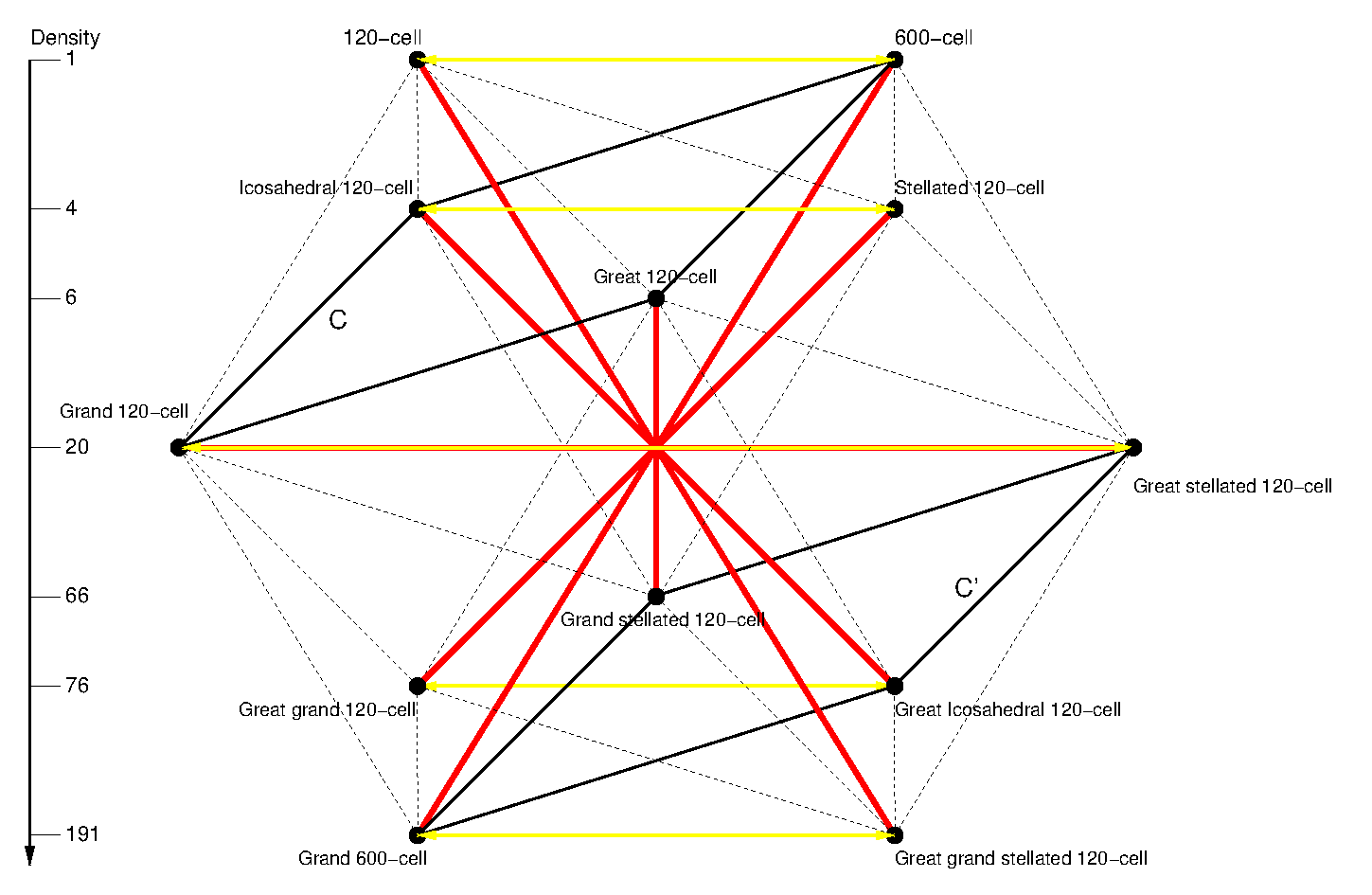Fig. 26a: Instead of 6 polyhedra shown in Figs. 4a and 4b, we now have 12 polychora, in this and the following plots we arrange them as the vertices of a Cuboctahedron, which has 12 vertices.
As in those earlier figures, the vertical scale indicates the density and the two-sided yellow arrows denote duality. Note that the Great 120-cell and the Grand stellated 120-cell are self-dual.
As in Fig, 4a, the red lines that go though the center of the arrangement change a pentagon into a pentagram, or vice-versa, either in the faces or in the vertex figures of the polychora at both ends. Thus the polychora connected by these red lines are isomorphic. This means that they are topologically identical: they have the same number of cells, faces, edges and vertices, the cells have identical numbers of faces, which have identical numbers of sides, the vertices have the same number of cells, faces and edges converging into them, etc.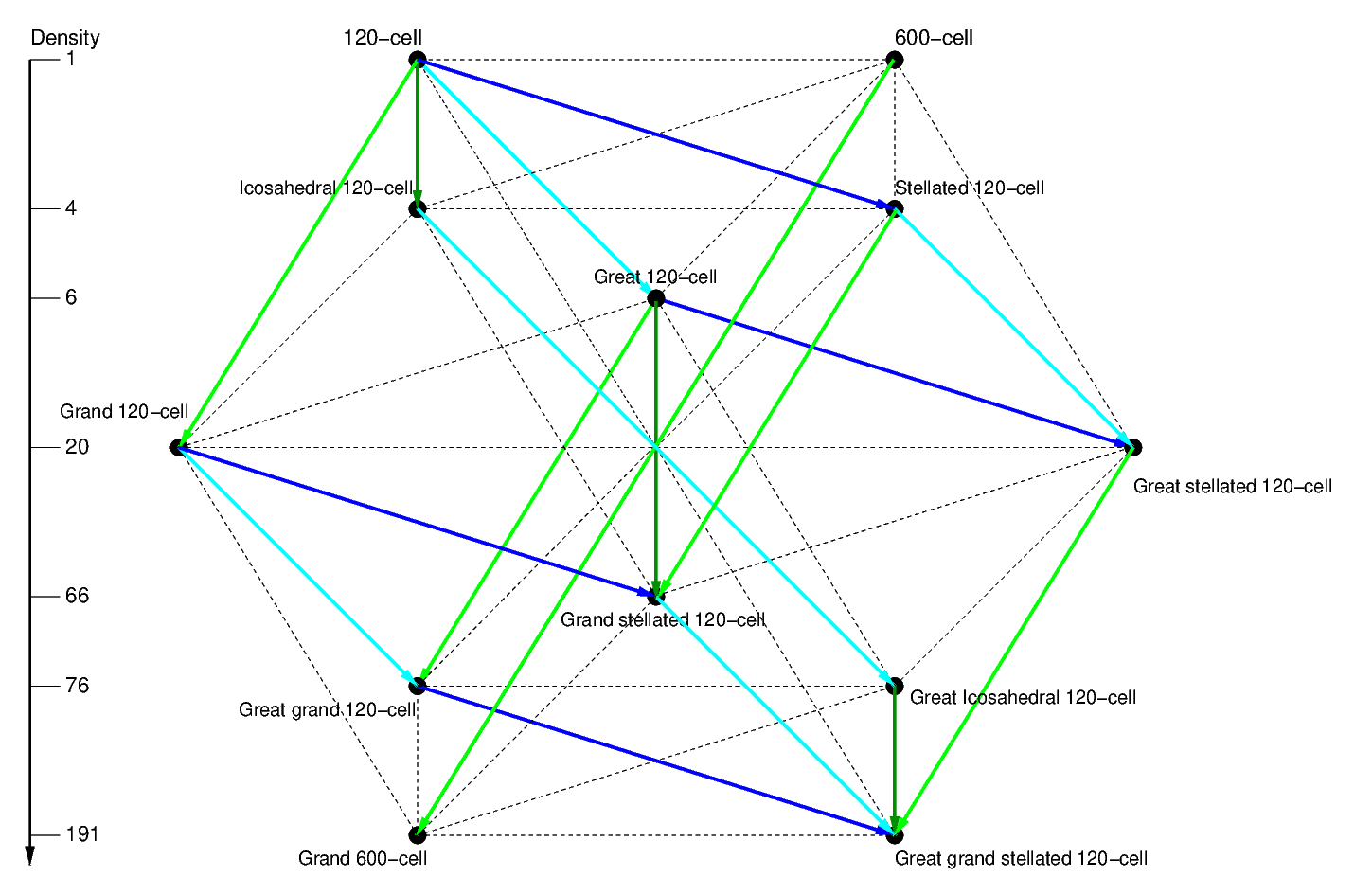Fig. 26b: With this cuboctahedral arrangement, many other geometric relations can be represented as sets of parallel lines, as in Fig. 4b. In this Figure we show four stellation operations, which give the Schläfli-Hess polychora their names. This figure follows a similar one presented by Conway, Burgiel and Goodman-Strauss (see references below).

1) Expansion of the 1-D elements. The dark blue arrows (the only among stellations that go diagonally on the square faces of the cuboctahedral arrangement, not along its edges or through its center) denote edge stellation, which has the same meaning as in 3-D, i.e., a finite extension of the edges along the same direction. In the case of the 120-cell, this operation produces the Small stellated 120-cell. Each of the 120 Dodecahedra of the 120-cell was edge-stellated into a Small stellated dodecahedron (Fig. 3); the latter has only 12 vertices (one for each face of the Dodecahedron) arranged as in the Icosahedron, with a pentagonal vertex figure. Likewise, the Small stellated 120-cell has only 120 vertices - one for each cell of the 120-cell, arranged as in the 600-cell, the vertex figure is a regular polyhedron with pentagonal faces - the Dodecahedron.

2) Expansion of the 2-D elements. The light blue arrows denote greatening; as in 3-D this means replacing a face by an identical, larger one in the same plane. Applying this operation to the 120-cell leads to the Great 120-cell, where each Dodecahedron of the 120-cell was greatened to a Great dodecahedron. Edge-stellating the Great 120-cell, or greatening the Small-stellated 120-cell, we obtain the Great stellated 120-cell, where each Dodecahedron of the 120-cell was tranformed into a Great stellated dodecahedron. The pattern of cell stellation in the top blue circuit is exactly the one shown in Fig. 11b. Greatening the Icosahedral 120-cell means that the cells of the resulting Great icosahedral 120-cell are the only regular stellation of the Icosahedron, i.e., Great icosahedra.

3) Expansion of the 3-D elements. I. The light green arrows denote a new operation for polychora, aggrandizing (replacing cells by identical larger ones in the same 3-d ``plane''). From this, we see that the cell types in the lower blue circuit will be a repeat of the cell types in the top blue circuit we just described. We also see from this that the Grand 600-cell also has Tetrahedra as cells, like the 600-cell.

4) Expansion of the 3-D elements. II. The dark green arrows denote anotther new operation for polychora, dual aggrandizing. In this operation, the cells are replaced by larger dual cells in the same 3-D ``plane''. Thus the Icosahedral 120-cell (the dual of the Small stellated 120-cell) will have 120 Icosahedra as cells.

Analogously to the 3-D case, none of these operations changes the number of cells or their 3-d ``planes''. Therefore, all stellations of the 120-cell must have 120 cells as well. The Grand 600-cell is the only stellation of the 600-cell, so it is the only Schläfli-Hess polychoron with 600 cells. This makes it the 4-d equivalent of the Great icosahedron in Fig. 3.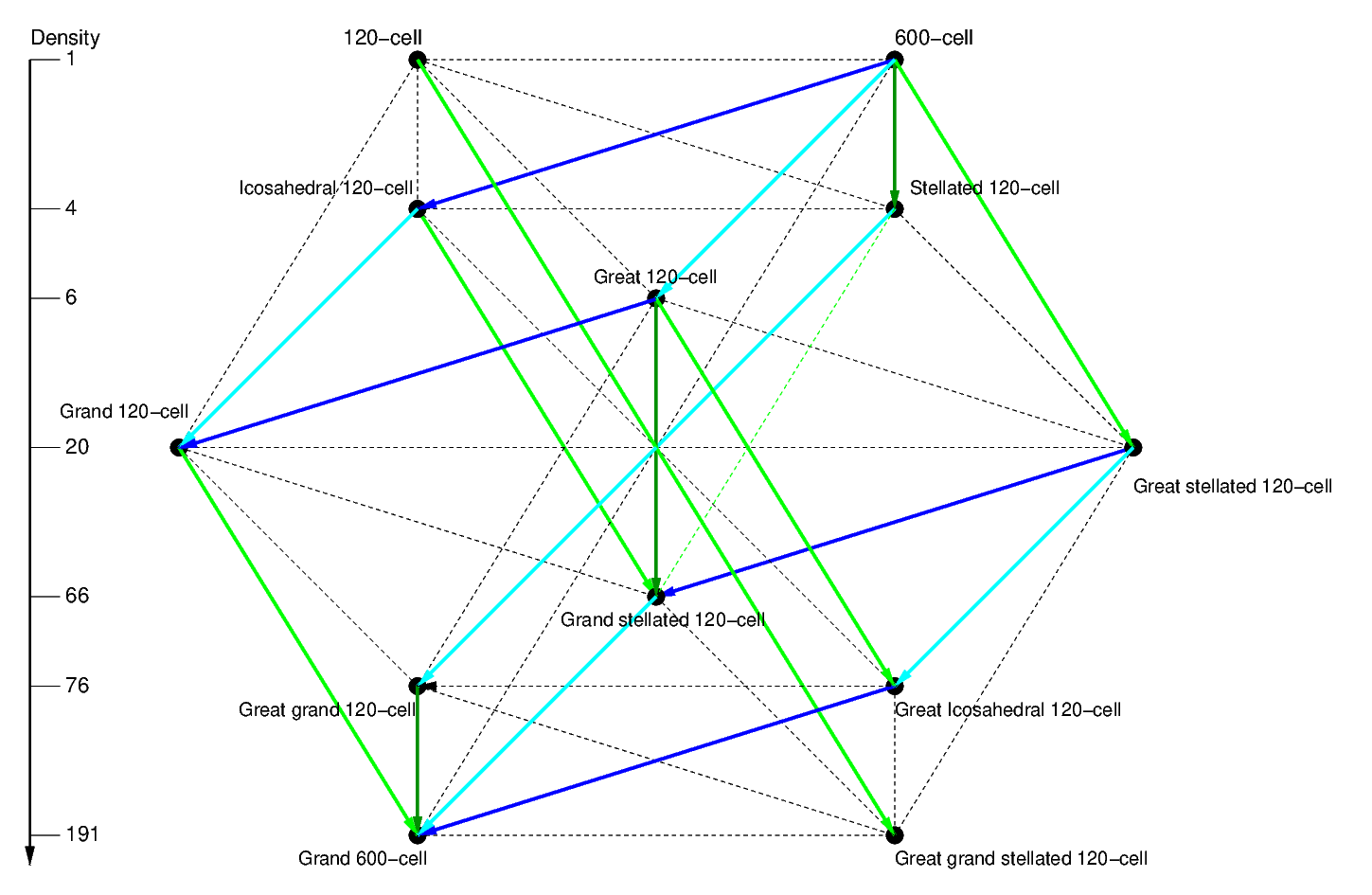Fig. 26c: Faceting relations. As in Fig. 4b these operations can be seen as the duals of the stellation operations in Fig. 26b, i.e., they are like a mirror image of the latter.

Since none of the stellation operations changes the number and hyperplane of the cells, all faceting operations preserve the vertex arrangements, as in 3 dimensions. Therefore, we see straight away that the nine polychora connected to the 600-cell have its 120 vertices. The Great grand stellated 120-cell, one of the many stellations of the 120-cell, is the only regular faceting of the 120-cell, i.e., it is the only Schläfli-Hess polychoron with 600 vertices. This makes it 4-D analogue of the Great stellated dodecahedron (see Fig. 3), which it has as cells.

We now describe the individual faceting operations.

1) Faceting with preservation of 2-D elements. The solid red and gold lines are equivalent to: finding dual + edge stellating + finding dual. Like its dual transformation, these are the only faceting relations represented as diagonals of squares of the cuboctahedral arrangement. Since the edge stellation preserves the edge directions, the dual faceting operation preserves their perpendicular face arrangements, which also imply the same edge arrangement.

2) Faceting with preservation of 1-D elements. The dashed lines are equivalent to find dual + greaten + find dual. Since greatening preserves the type of face and its plane, the dual faceting operation preserves their perpendicular edge arrangement.
Since Zometool models represent edge arrangements, this conservation law is what defines how the polychora are represented: The 4 polychora in the red circuit (all that can be reached from the 120-cell along the edges of the cuboctahedral arrangement) are represented by the model of the 600-cell (Fig. 18), the 2 polychora in the dashed brown line are represented by the model of the Small stellated 120-cell (Figs. 23a and b) and the 4 polychora in the gold circuit are represented by the model of the Grand 600-cell (Figs. 24a and b). The Zometool models of the 120-cell (Fig. 19) and the Great grand stellated 120-cell (Figs 25a and b) represent only those polychora.

These two operations do not merely preserve face or edge arrangements. They facet the vertex figure, a consequence of the fact that their dual operations stellate the cells. In a way analogous to the two faceting operations described in Fig. 4b, the first operation facets the vertex figure preserving its edges, the second operation facets the vertex figure by reducing the angular size of its (2-D) vertex figure, which otherwise remains unchanged.
Thus, since the 600-cell has an Icosahedron as a vertex figure, the other 3 polytopes in the red circuit will have as their vertex figures the 3 facetings of the Icosahedron shown in Fig. 11c: The Icosahedral 120-cell has the Great dodecahedron as vertex figure, the Great 120-cell has the Small stellated dodecahedron as vertex figure, and the Grand 120-cell has the Great icosahedron as vertex figure.
Since the Small stellated 120-cell has a Dodecahedral vertex figure, its regular faceting, the Great grand 120-cell must have the only regular faceting of the Dodecahedron, the Great stellated dodecahedron, as a vertex figure. The dodecahedral vertex figure of the Small stellated 120-cell admits many more facetings (see Fig. 12d), this results in several other polychora and compounds below (see Fig. 23c) which have the same edge arrangement, and therefore the same Zometool representation.

3) Faceting with preservation of 0-D elements. I. The solid blue lines are equivalent to: find dual + aggrandize + find dual. This type of faceting only preserves the vertex arrangement, the bare minimum for any faceting operation. However, since aggrandizing preserves the cell type but enlarges it, the dual faceting operation preserves the type of vertex figure, but reduces its angular size. The light blue lines show that each polychoron in the red family has a faceting in the yellow family with the same type of vertex figure as those we described above. The dark blue line shows that the only faceting of the 120-cell, the Great grand stellated 120-cell, also shares the former's vertex figure, the Tetrahedron.

4) Faceting with preservation of 0-D elements. II. The solid brown lines are equivalent to: find dual + dual aggrandize + find dual. This type of faceting also preserves only the vertex arrangement. However, since dual aggrandizing preserves the cell plane but replaces the cells by larger dual cells in the same 3-D plane, the dual faceting operation transforms the vertex figure into its dual, while reducing its angular size.

Now, a final note regarding the methods of construction of the models of the Grand 600-cell (Figs. 24a and b) and Great grand stellated 120-cell (Figs. 25a and b). As we can see in Fig. 26b, the Great stellated 120-cell and the Grand stellated 120-cell (which have the same edge arrangement as the Grand 600-cell, see Fig. 26c) can be obtained as edge stellations of the Great 120-cell and the Grand 120-cell respectively (which have the same edge arrangement as the 600-cell, see Fig. 26c). Therefore we can obtain the edge arrangement of the Grand 600-cell by extending the edges of the 600-cell until they meet again in a larger set of vertices of the 600-cell - which are also the vertices of the Grand 600-cell.
Also, as we see in Fig. 26b, the Great grand stellated 120-cell can be obtained by edge-stellating the Great grand 120-cell - which as we have seen in Fig. 26c, has the same edge arrangement as the Small stellated 120-cell. Thus one obtains the edge arrangement of the Great grand stellated 120-cell by extending the edges of the Small stellated 120-cell until the meet at the vertices of a larger 120-cell, which are also the vertices of the Great grand stellated 120-cell.

Before ending this section, it is important to note that the convex uniform and the regular non-convex polychora represent only a tiny fraction of the 1849 known uniform polychora, the vast majority of them non-convex. No hope of making models of all of these! However, we have mentioned already some of these strange objects above (in the caption of Fig. 20); which are represented by the same wire frame of the Rectified 600-cell. We will find a few more that are represented by another model above, that of the Small stellated 120-cell.

## Regular polychoron compounds

``Nature uses as little as possible of anything.''
Johannes Kepler

Before starting this section, we must present a brief explanation of the concept of Triality.

In the case of duality in 3 dimensions, one can, by the definition of the meaning of duality, scale two concentric dual polyhedra in such a way that each edge from a polyhedron meets another (perpendicular) edge from the dual polyhedron at a point that is in the middle of both (the midpoint). Those two edges form a plane that is perpendicular to a third line joining the midpoint to the center of those polyhedra. Rectifying those two dual polyhedra results in the same rectified polyhedron.
In the case of Triality in 4 dimensions, the situation is very similar, except that the perpendicular edges of three concentric ``trial'' polychora (16-cells for the only regular case) meet at the midpoint. These edges are in a 3-D ``plane'' that is itself perpendicular to a fourth direction, the line joining the midpoint to the centers of the trial polychora. Rectifying all three polychora results in the same rectified polychoron, the regular case being the 24-cell, whose vertices coincide with the previous midpoints.

The same applies to projections: if we pick three ``trial'' projections of the 16-cell and rectify them, we obtain an invariant projection of the 24-cell. In this page, we show a triad of such trialities. The first we met already in Figs. 15a and 15b, triality is the reason for the identical rectifications of the vertex-first and cell-first projections of the 16-cell. The other two are shown in Figs. 27a and 27b.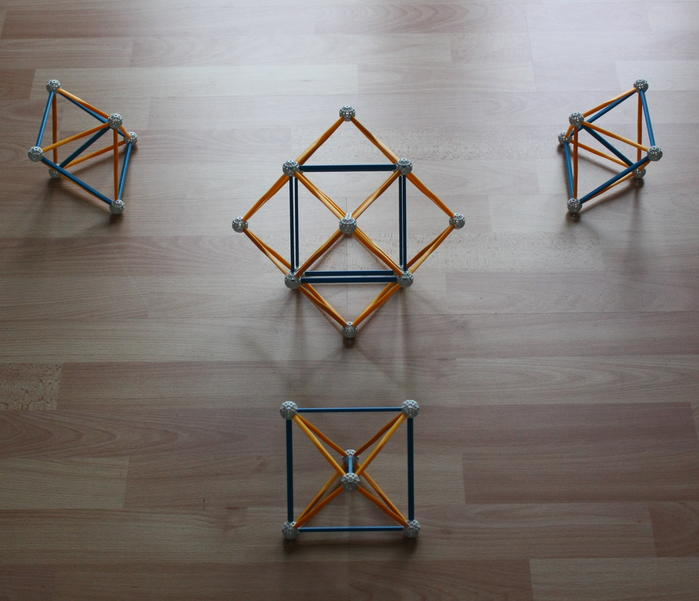Fig. 27a: Three edge-first projections of the 16-cell, with the central edges of the projections loosely aligned along 3 perpendicular orthogonal axes in 3-d space. Rectifying all three projections results in the same projection of the 24-cell, which is shown in the center. This is its vertex-first (or ``pyritohedric'') projection, the dual of the cell-first projection in Figs. 15a and 15b.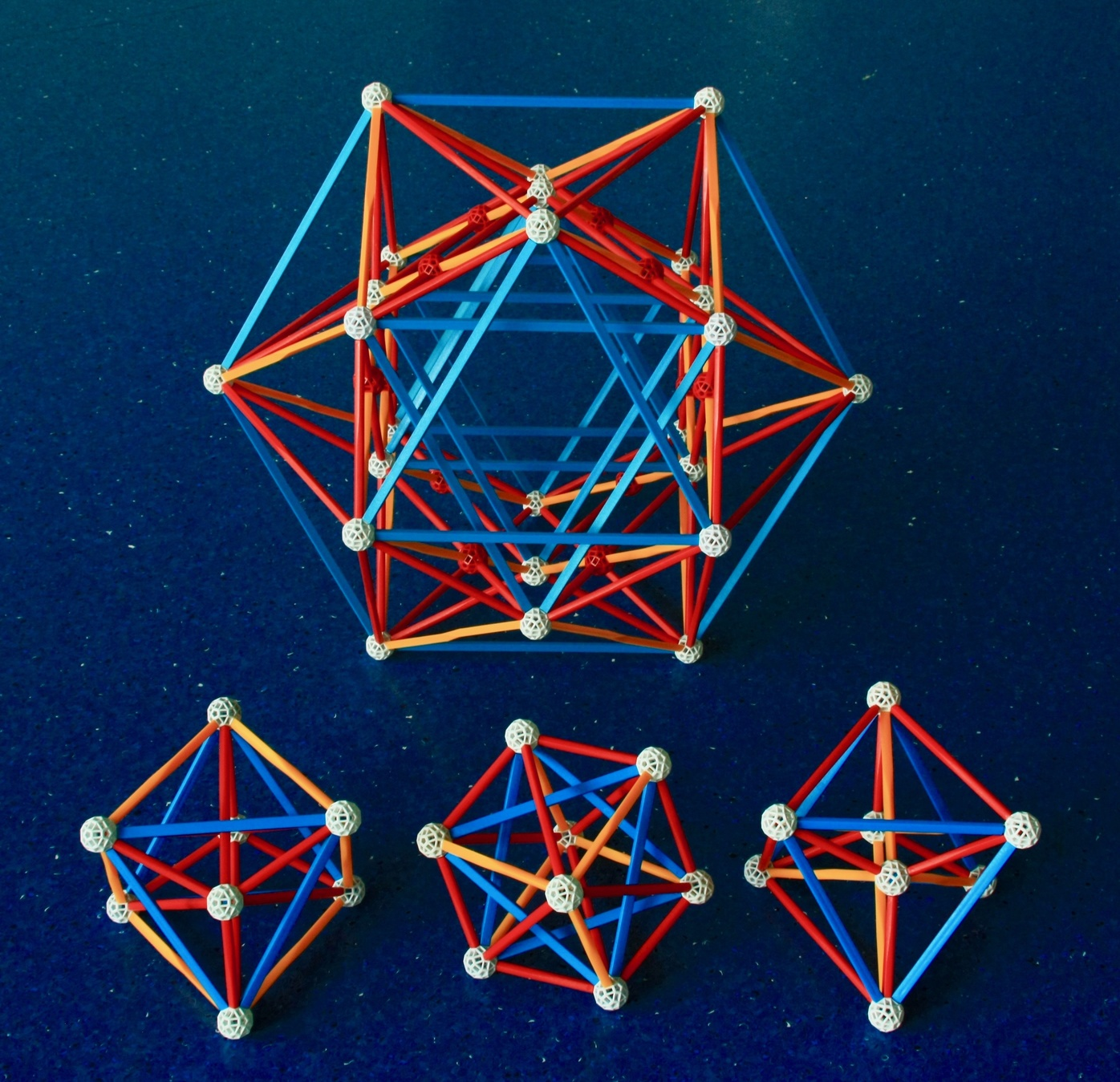Fig. 27b: Bottom: The three ``prismatic'' projections of the 16-cell. Rectifying all three projections results in the same projection of the 24-cell, also known as its ``prismatic'' projection, this is shown by the larger model above. In that model, the real vertices are represented by the white balls, the red balls represent edge intersections that happen only in the 3-d projection. One of the beauties of these models is that they show all vertices, edges, faces and cells separately, without superpositions.
For a detailed explanation of these models, see David Richter's Triality with Zometool page.

After this explanation, we now move to the regular polychoron compounds. As in the case of the regular polyhedron compounds, these can be seen as important facetings of the regular polytopes. They also illustrate the fact that some of the polychoron symmetry groups are sub-groups of others.

The concept of triality allows a better understanding of the first object we discuss below, the compound of three 16-cells. We present three projections of this compound, which result directly from stellating (and/or faceting) the three projections of the 24-cell in Figs. 16, 27a and 27b.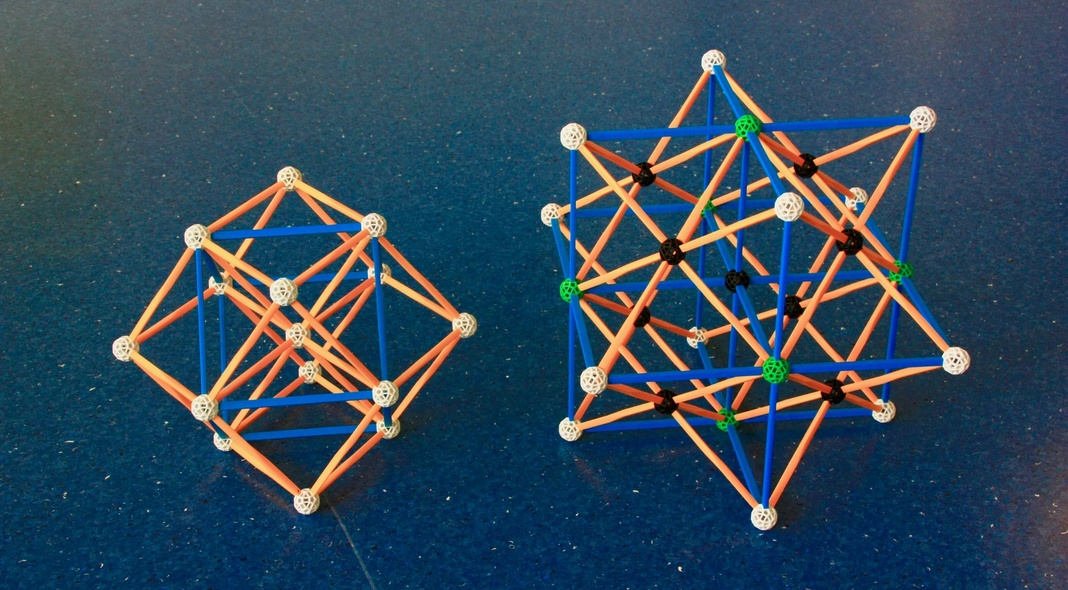Fig. 28a: Superposing the 3 orthogonal 16-cells in Fig. 27a, we obtain, on the right, a regular polychoron compound with three 16-cells. This is the first stellation of the 24-cell; this particular projection results from stellating the pyritohedric projection of the 24-cell on the left. Since the latter has the Rhombic dodecahedron as its outer envelope, this projection has, as its outer envelope, the first stellation of the Rhombic dodecahedron; the latter is also known as ``Escher's polyhedron''.

Each of the vertices of the 24-cell is transformed into the mid-point of three edges of the compound of 3 16-cells (remember the triality!), these are represented in this and the following figures (28b and 28c) by the black balls, the real vertices are represented in these figures by the white balls. The green balls in this model represent 3-edge intersections that coincide with a real vertex of the 16-cells, this happens only in this model.
An inspection of the model on the right will show that the real vertices have the same arrangement as the vertices of the cell-first projection of the 24-cell (seen on Fig. 28b on the left), which is the dual of the pyritohedric projection of the 24-cell. This implies that (as mentioned in the caption of Fig. 16) the vertices of the regular compound of three 16-cells are arranged as the vertices of a 24-cell.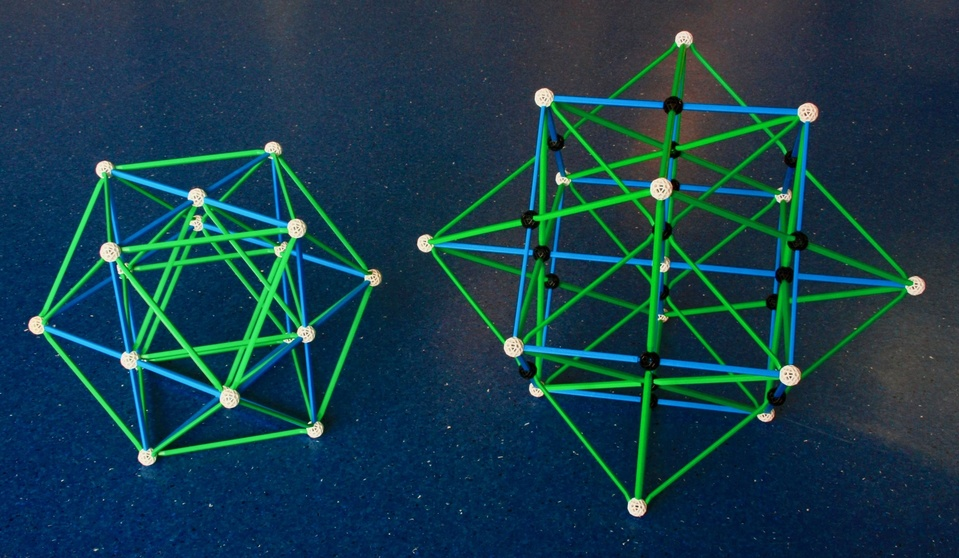Fig. 28b: Combining one vertex-first projection of the 16-cell in Fig. 15a with two complementary superposed cell-first projections of the 16-cell in Fig. 15b, one obtains, on the right, a different projection of the compound of three 16-cells. This projection is the stellation of the cell-first projection of the 24-cell on the left. Since the latter has a Cuboctahedral envelope; its stellation's envelope is a composite of a Cube and a Octahedron. The real vertices of this projection coincide with those of of the pyritohedric projection of the 24-cell (on the left in Fig. 28a), but have, in this model, twice the separation. This means that although the dual of the 24-cell is also a 24-cell, one of the dual pairs is larger (or smaller) than the other by the square root of 2.

This model shows very clearly that each of the individual Octahedra of the 24-cell was stellated into a ``Stella Octangula'', as in Figs. 10a and b. Therefore, the compound of three 16-cells can also be seen as a compound of 24 ``Stella Octangula'', with a total of 48 Tetrahedral cells.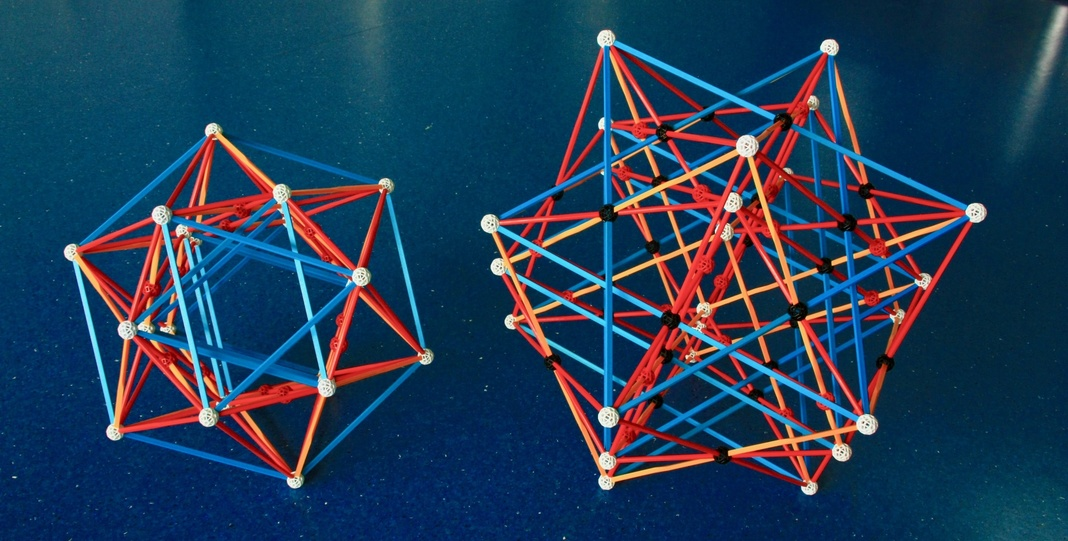Fig. 28c: Combining the three prismatic projections of the 16-cell in Fig. 27b one obtains, on the right, the prismatic projection of the compound of three 16-cells. This is the stellation of the prismatic projection of the 24-cell on the left, which also appeared in Fig. 27b. The red balls in both models are edge intersections that appear because of the projection; they are not real edge intersections in 4 dimensions. The real vertices of the compound of three 16-cells (white balls) have the same arrangement of the dual of the prismatic projection of the 24-cell, a projection of the 24-cell that cannot be built in the Zometool system. Both models are built using very long struts, which are no longer sold by Zometool.

How to build: Check David Richter's dedicated page, which also provides many more details on this model.

One of the advantages of the prismatic projection of the compound of three 16-cells is that is shows all 48 real and false vertices of the compound separately - no superposed vertices here. All edges, faces and cells are also shown in the model. These real and false vertices are the same as the vertex arrangement of the dual configuration of 24-cells; this represents the 48 root vectors of the F4 group. Like the 24-cell, this is a truly exceptional object.Fig. 28d: On top right we see the pyritohedric projection of the compound of 3 16-cells. On lower right, we see one of the components of the latter, the edge-first projection of the 16-cell. Its dual is the face-first projection of the Tesseract (lower left).
The dual of the compound of three 16-cells is another regular compound, with three tesseracts. Amazingly, a projection of that regular compound (the dual of the pyritohedric projection of the compound of 3 16-cells) is well represented by the cell-first projection of the 24-cell (above left). With some attention, you might discern the 3 face-first Tesseracts in that model. The 3 Tesseracts have a total of 16 × 3 = 48 vertices, this is twice as much as the 24 vetices of the 24-cell, this implies that each vertex of the 24-cell is shared by two Tesseracts. The vertex figure of this compound therefore consists of two vertex figures of the Tesseract, the Tetrahedron. Thus the vertex figure of this compound is the regular compound known as Stella Octangula (Fig. 10b).

Thus all Zometool projections of the 24-cell (including those in Figs. 28a, b and c) are also projections of the compound of 3 Tesseracts. As an example, the pyritohedric projection of the 24-cell looks exactly like a superposition of two complementary vertex-first and one face-first projections of the Tesseract in Figs. 15a and 15b.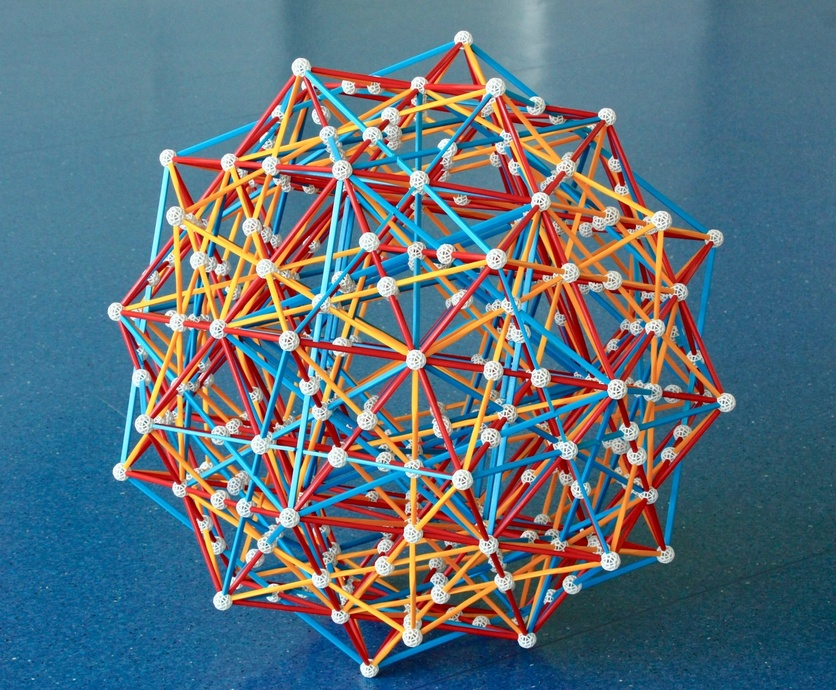Fig. 29a: With five 24-cells (in this projection one is in pyritohedric projection, as in Fig. 27a, and four are in prismatic projection, as in Fig. 27b), we obtain a self-dual regular polychoron compound. In this picture, we're looking at its 3-d projection through a genuine three-fold symmetry axis. This compound has 5 × 24 = 120 vertices, which happen to have the same arrangement as the 120 vertices of the 600-cell in Fig. 18. This illustrates the fact mentioned in the caption of that figure that the vertices of the 600-cell can be decomposed in five sets, each with the same arrangement as the vertices of a 24-cell. The edges of this model are a sub-set of the edges of the Small stellated 120-cell in Figs. 23a and 23b.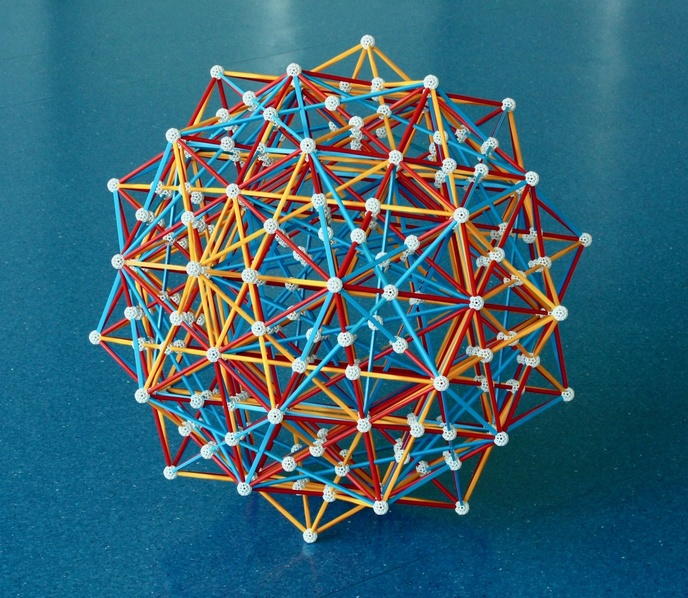Fig. 29b: The regular compound of five 24-cells, now seen through a five-fold ``Ghost symmetry'' axis.

Note that since the edges and vertices of the compound of three Tesseracts coincide with those of a 24-cell, the model above also represents another regular compound, one with 15 Tesseracts. In that case, each of the 120 vertices is shared by two Tesseracts, instead of one 24-cell. The vertex figure is then the same as for the Compound of 3 Tesseracts, the regular compound of two Tetrahedra, the Stella octangula.

See detailed explanation of this model in David Richter's dedicated page, which includes a part count. Here we're building this model on a φ smaller scale than the model in David Richter's page. This has an interesting implication: If we can find some of the very long old struts no longer manufactured by Zometool (12 very long reds, 12 very long yellows), we can use each of them to replace a combination of two struts and one ball where the ball is not strictly necessary (i.e., a ball where there is no intersection with other edges). Doing this, we can save 48 regular-sized struts and 24 balls compared to the part count in David Richter's page, and improve the appearance of the model.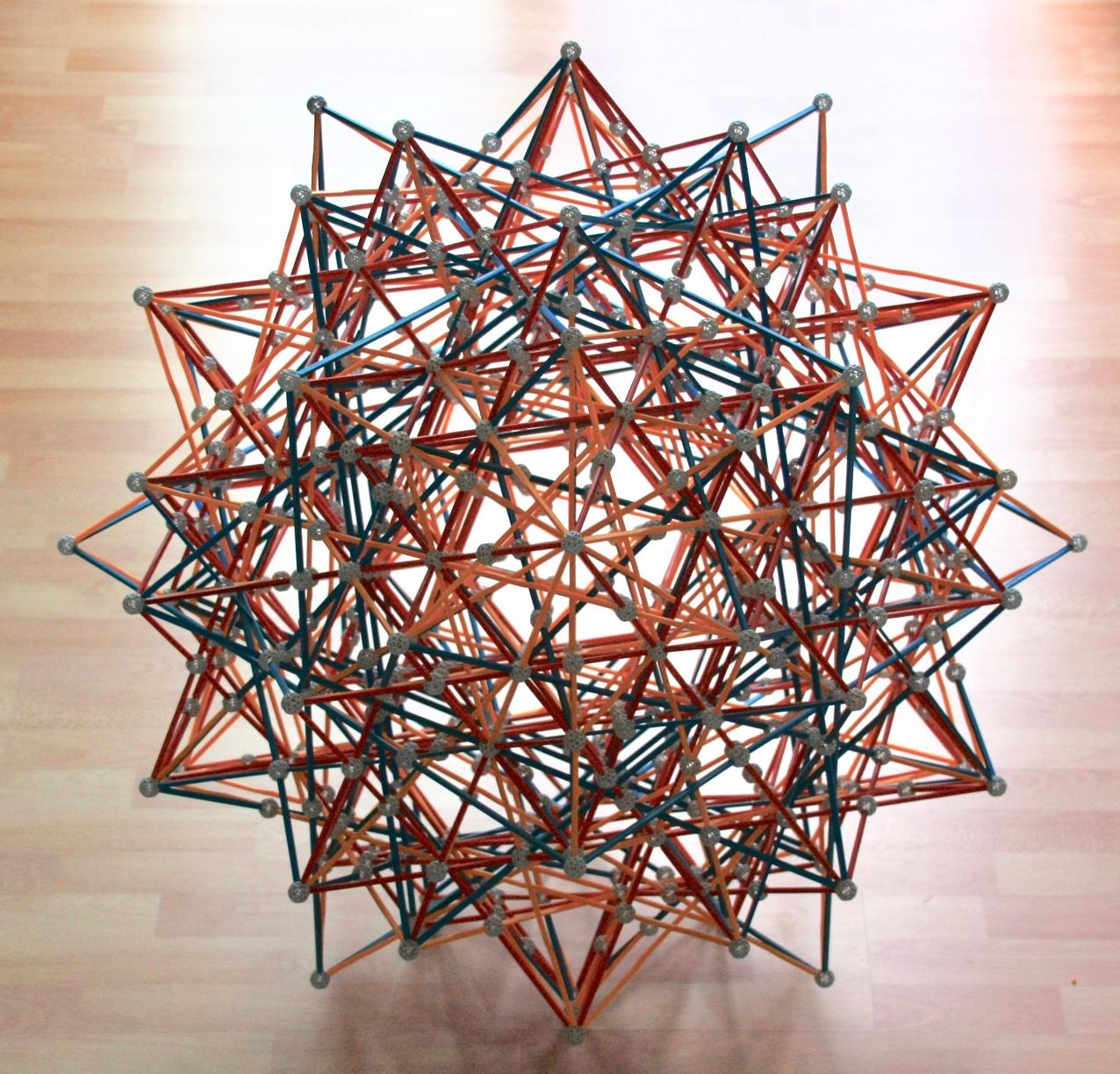Fig. 30a: Combining the pyritohedric projection of the compound of 3 16-cells (in Fig. 28a) with four prismatic projections of the compound of 3 16-cells (in Fig. 28c), we obtain a projection of the dual of the compound of 15 Tesseracts in Figs. 29a and b, the (also regular) compound of fifteen 16-cells. This is also the stellation of the compound of five 24-cells in Figs. 29a and b. Here we see it through the 3-fold symmetry axis.

Each compound of 3 16-cells has the same vertices as a 24-cell, thus the vertices of this set of 15 16-cells (arranged in 5 groups of 3 16-cells) are arranged as the vertices of the compound of 5 24-cells, which is the same vertex arrangement of the 600-cell. Interestingly, in this projection the vertices are not arranged as the vertices of the projection of the 600-cell in Fig. 18, but as a sub-set of the 600 vertices of the 120-cell in Fig. 19. This compound can also be seen as a compound of 120 ``Stella Octangula''.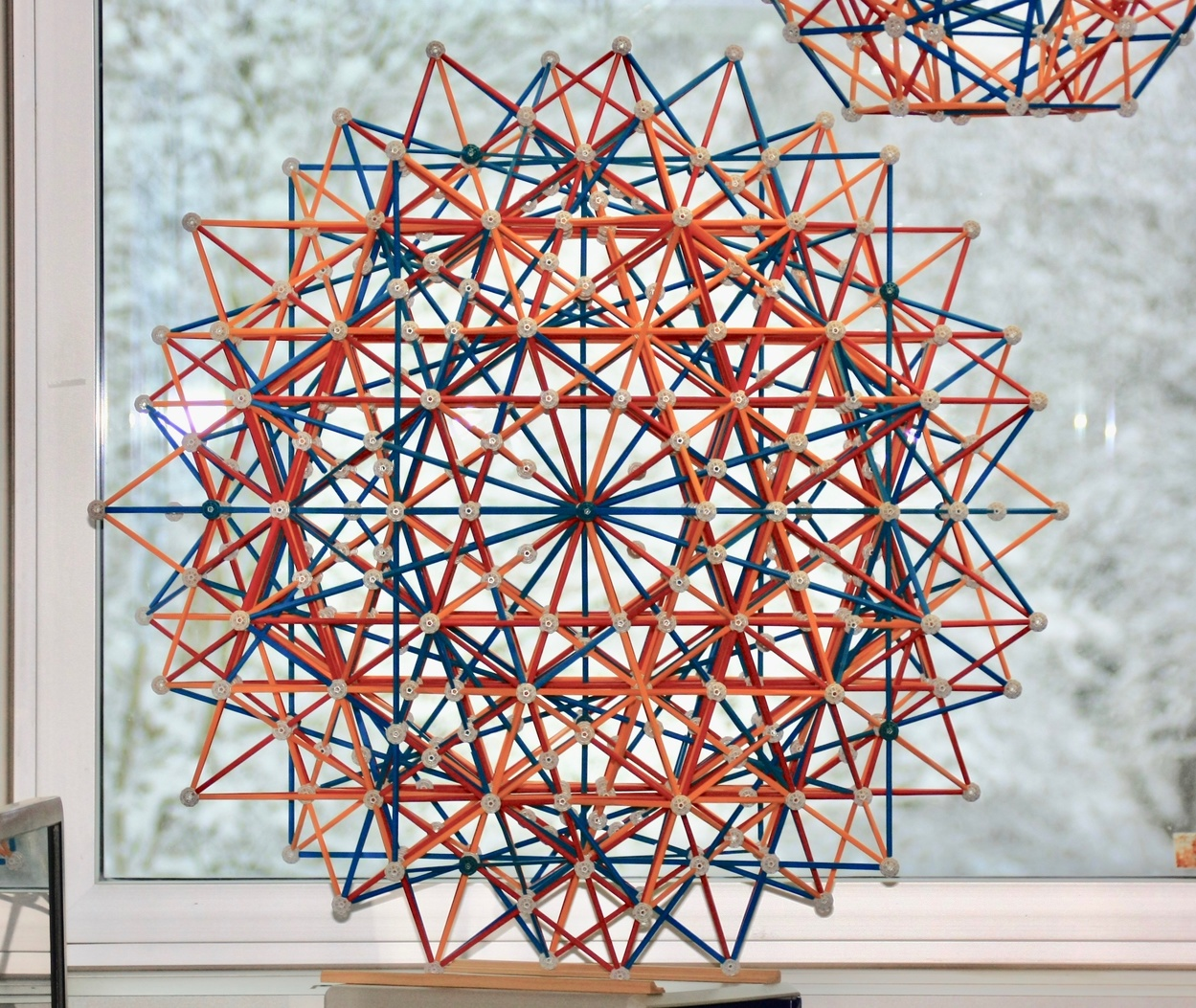Fig. 30b: The compound of 15 16-cells, here seen through a 5-fold ``ghost symmetry'' axis.
See also David Richter's dedicated page, which has a detailed description and part count. As in the model of the compound of 5 24-cells, if we can find some of the very long old struts (in this case, 48 very long reds, 120 very long yellows and 72 very long blues), we can save 480 regular-sized struts and 240 balls compared to the part count in David Richter's page, and greatly improve the appearance of the model.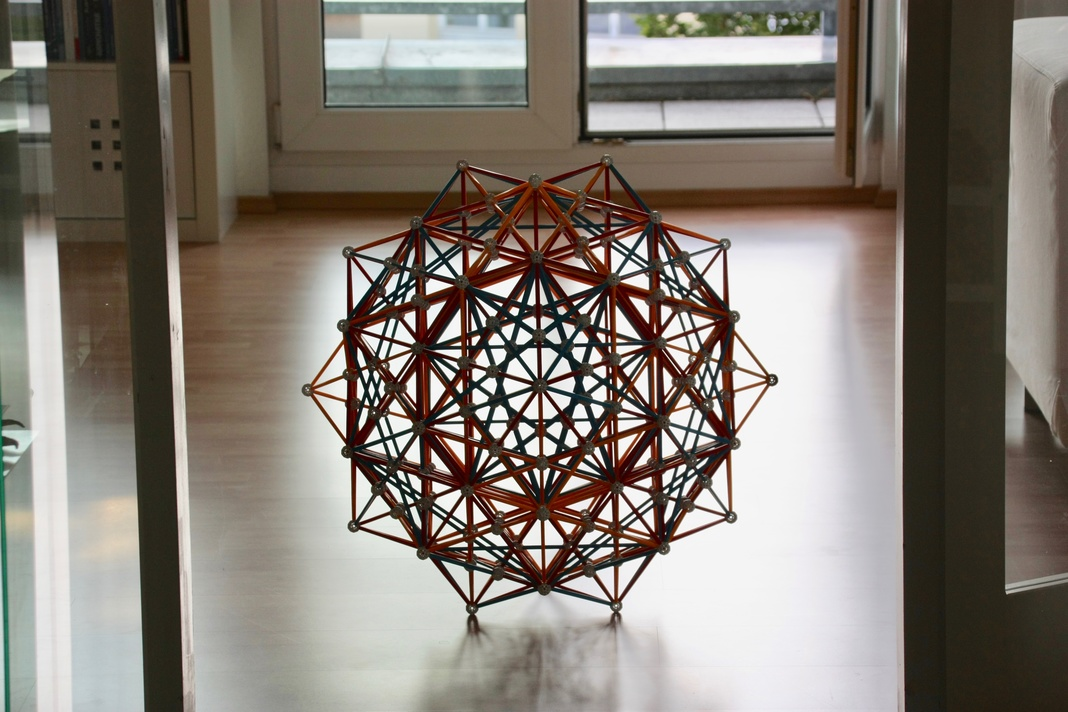Fig. 29c: Rectifying the compound of 15 16-cells, we produce three superposed copies of the compound of 5 24-cells. As in Fig. 29b, we're looking at the model through one of its ghost symmetry axes. However, in this photo the strut colors are not as obvious, so the model resembles even more the model of the Small stellated 120-cell in Figs. 23a and 23b.

As remarked above, the edges of this model are a sub-set of the edges of the Small stellated 120-cell in Figs. 23a and 23b, while the vertices have the same arrangement as the latter (which are the same as the 600-cell). For each axis of ghost symmetry, we can superpose four other sets of five 24-cells, each set rotated relative to the previous by 72 degrees around that axis. The result is in the picture below: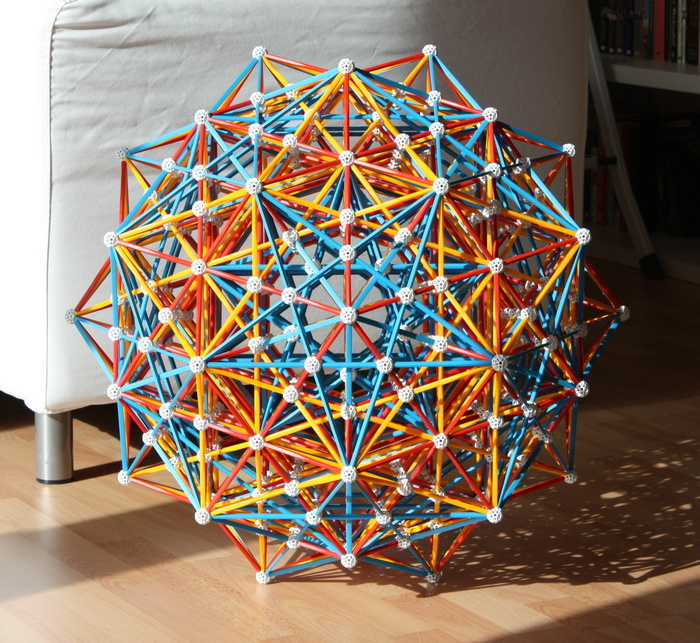Fig. 23c: There is a pair of dual compounds of 25 24-cells, one of them a faceting of the 120-cell and the other a faceting of the 600-cell. The model above represents the latter. Since the 600 vertices of the 25 24-cells must appear in the positions of the 120 vertices of the 600-cell, each vertex must be shared by five different 24-cells. The 25 × 96 = 2400 edges will twice cover be the full set of 1200 edges of the Small stellated 120-cell, i.e., each edge of the Small stellated 120-cell is shared by two 24-cells. Thus the model of this compound is identical with that of the Small stellated 120-cell in Figs. 23a and 23b!
Therefore, this compound can be understood as an edge-preserving faceting of the Small stellated 120-cell. In the caption to Fig. 26b and c, we remarked that the latter has a Dodecahedral vertex figure. This can be faceted in a number of ways, one of them is as a Compound of 5 cubes. That is the vertex figure of this Compound of 25 24-cells: the cube is the vertex figure of the 24-cell, and as we just said, each vertex is shared by 5 24-cells.

Again, because the edges and vertices of the 24-cell coincide with those of the compound of three Tesseracts (with each vertex shared by two Tesseracts), the edges of the two compounds of 25 24-cells mentioned above coincide with those of two different compounds of 75 Tesseracts. Thus the Zometool model above also represents one of those two, the regular compound of 75 Tesseracts that is also a faceting of the 600-cell and an edge-preserving faceting of the Small stellated 120-cell. Each of the 120 vertices of this compound is shared by 10 Tesseracts. Thus the vertex figure of this compound is the Compound of 10 Tetrahedra (the latter being the vertex figure of the Tesseract). The Compound of 10 Tetrahedra is also a faceting of the Dodecahedral vertex figure of the Small stellated 120-cell.

This ends the list of the 4 regular facetings of the Small stellated 120-cell.

As a side curiosity, the Dodecahedral vertex figure of the Small stellated 120-cell can be faceted in many other ways. This results in an additional 14 uniform non-convex polychora, all edge-preserving facetings of the Small stellated 120-cell and therefore represented by the same Zometool model.
Among these, there are three semi-regular polychora, which result from faceting that Dodecahedral vertex figure as the three ditrigonal quasi-regular star polyhedra in Fig. 6. The latter have the same edge arrangement as the Compound of five cubes - the vertex figure of the Compound of 25 24-cells - therefore these three non-convex semi-regular polychora have the same face arrangement as the latter compound:
1) If the vertex figure faceting is the Small ditrigonal icosidodecahedron, we obtain the Small ditrigonary 120-600-cell, which has 120 Great icosahedra and 600 Tetrahedra as cells (this name is consistent with those of the quasi-regular polyhedra, for which the names indicate the number of the two types of faces separately).
2) If the vertex figure faceting is the Ditrigonal dodecadodecahedron, then we obtain the Ditrigonary 120-120-cell, which has 120 Great icosahedra and 120 Icosahedra as cells.
3) Finally, if the vertex figure faceting is the Great ditrigonal icosidodecahedron, then we obtain the Great ditrigonary 120-600-cell, which has 120 Icosahedra and 600 Tetrahedra as cells.

With relatively few models, we have built projections of seven of the regular polychoron compounds:
• Three different projections of the compound of 3 16-cells (Figs. 28a, b and c). These are at the same time facetings and stellations of the 24-cell,
• Three different projections of the compound of 3 Tesseracts (identical to the three projections of the 24-cell in Figs. 28a, b and c). This is the dual of the compound of 3 16-cells. Also a faceting of the 24-cell,
• The self-dual compound of 5 24-cells (Figs. 29a, b and c),
• The compound of 15 Tesseracts (identical to model of the compound of 5 24-cells in Figs. 29a, b and c),
• The compound of 15 16-cells (see Figs. 30a and b), this is the dual of the previous compound of 15 Tesseracts and a stellation of the compound of 5 24-cells,
• One of the two dual compounds of 25 24-cells (identical to the model of the Small stellated 120-cell, see Fig. 23c), a faceting of the 600-cell, and
• One of the two compounds of 75 Tesseracts (identical to the model of the compound of 25 24-cells, see also Fig. 23c), this one also a faceting of the 600-cell.
This is by no means the whole list: there are, in total, 32 regular polychoron compounds. The nice thing about this list, though, is that, together with nine of the 10 non-convex regular polychora, it completes all regular facetings of the 600 cell that can be built with the Zometool system (one of the facetings of the 600-cell, one of the two compounds of 75 16-cells, cannot be built with the Zometool system, but it is very similar to the compound of 15 16-cells, in the same way that the compound of five Tetrahedra is similar to the compound of ten Tetrahedra: the first already covers the vertices uniquely).

Some of the other compounds can also be built with Zometool. One example is one of the two compounds of 75 16-cells (see David Richter's page on that compound). This can be obtained from the compound of 15 16-cells using the same method we used to derive the compound of 25 24-cells from the compound of 5 24-cells: make 4 more copies and rotate them around the ghost symmetry axis. Therefore looking at the projection of the compound of 75 16-cells from its real 5-fold symmetry axis will be similar to the view of the projection of the compound of 15 16-cells along its ghost symmetry axis (shown in Fig. 30b). Unlike most compound models we have shown above, that latter is a faceting of the 120-cell.

I might do that model some other time. For now, it's time to go beyond the fourth dimension.

## References

Here are a few great books where you can find the truth about polyhedra, polytopes and how to build them:
... and here are a few nice sites:
• The most valuable resource for anyone in the first stages of learning any topic must surely be Wikipedia, one of the best things to happen in the Internet age. I link to it extensively in this page.

• David Richter's page, which includes a very nice list of Zometool projects. Many of the models in my office (600-cell, first stellation of the 120-cell and others) were built following directly the instructions in these pages - I learned a lot building those models! Most others were inspired by models that appear in these pages. A big Thank You to David for the very inspiring and educational resource!

• George Hart's page. From his beautiful site and its links you may explore the whole polyhedral universe.

• Eusebeia 4-D visualization. This is very useful for figuring out how to build Zometool projections of 4-D regular and uniform polychora.

• Uniform Polychora and Other Four Dimensional Shapes. This is an amazing site, which lists all 1849 known uniform polychora. If new ones are discovered, this is the place to look for them.